ROOT   Reference Guide## Legacy Code

TSpectrum is a legacy interface: it is not recommended to use it in new code. There will be no bug fixes nor new developments.

This class contains advanced spectra processing functions for:

• One-dimensional background estimation
• One-dimensional smoothing
• One-dimensional deconvolution
• One-dimensional peak search

The algorithms in this class have been published in the following references:

1. M.Morhac et al.: Background elimination methods for multidimensional coincidence gamma-ray spectra. Nuclear Instruments and Methods in Physics Research A 401 (1997) 113-132.
2. M.Morhac et al.: Efficient one- and two-dimensional Gold deconvolution and its application to gamma-ray spectra decomposition. Nuclear Instruments and Methods in Physics Research A 401 (1997) 385-408.
3. M.Morhac et al.: Identification of peaks in multidimensional coincidence gamma-ray spectra. Nuclear Instruments and Methods in Research Physics A 443(2000), 108-125.

These NIM papers are also available as doc or ps files from:

Definition at line 18 of file TSpectrum.h.

## Public Types

enum  {
kBackOrder2 =0 , kBackOrder4 =1 , kBackOrder6 =2 , kBackOrder8 =3 ,
kBackIncreasingWindow =0 , kBackDecreasingWindow =1 , kBackSmoothing3 =3 , kBackSmoothing5 =5 ,
kBackSmoothing7 =7 , kBackSmoothing9 =9 , kBackSmoothing11 =11 , kBackSmoothing13 =13 ,
kBackSmoothing15 =15
}Public Types inherited from TObject
enum  {
kIsOnHeap = 0x01000000 , kNotDeleted = 0x02000000 , kZombie = 0x04000000 , kInconsistent = 0x08000000 ,
}

enum  { kSingleKey = (1ULL << ( 0 )) , kOverwrite = (1ULL << ( 1 )) , kWriteDelete = (1ULL << ( 2 )) }

enum  EDeprecatedStatusBits { kObjInCanvas = (1ULL << ( 3 )) }

enum  EStatusBits {
kCanDelete = (1ULL << ( 0 )) , kMustCleanup = (1ULL << ( 3 )) , kIsReferenced = (1ULL << ( 4 )) , kHasUUID = (1ULL << ( 5 )) ,
kCannotPick = (1ULL << ( 6 )) , kNoContextMenu = (1ULL << ( 8 )) , kInvalidObject = (1ULL << ( 13 ))
}

## Public Member Functions

TSpectrum ()
Constructor. More...

TSpectrum (Int_t maxpositions, Double_t resolution=1)

~TSpectrum () override
Destructor. More...

virtual TH1Background (const TH1 *hist, Int_t niter=20, Option_t *option="")
One-dimensional background estimation function. More...

const char * Background (Double_t *spectrum, Int_t ssize, Int_t numberIterations, Int_t direction, Int_t filterOrder, bool smoothing, Int_t smoothWindow, bool compton)
This function calculates background spectrum from source spectrum. More...

const char * Deconvolution (Double_t *source, const Double_t *response, Int_t ssize, Int_t numberIterations, Int_t numberRepetitions, Double_t boost)
One-dimensional deconvolution function. More...

const char * DeconvolutionRL (Double_t *source, const Double_t *response, Int_t ssize, Int_t numberIterations, Int_t numberRepetitions, Double_t boost)
One-dimensional deconvolution function. More...

TH1GetHistogram () const

Int_t GetNPeaks () const

Double_tGetPositionX () const

Double_tGetPositionY () const

TClassIsA () const override

void Print (Option_t *option="") const override
Print the array of positions. More...

virtual Int_t Search (const TH1 *hist, Double_t sigma=2, Option_t *option="", Double_t threshold=0.05)
One-dimensional peak search function. More...

Int_t Search1HighRes (Double_t *source, Double_t *destVector, Int_t ssize, Double_t sigma, Double_t threshold, bool backgroundRemove, Int_t deconIterations, bool markov, Int_t averWindow)
Old name of SearcHighRes introduced for back compatibility. More...

Int_t SearchHighRes (Double_t *source, Double_t *destVector, Int_t ssize, Double_t sigma, Double_t threshold, bool backgroundRemove, Int_t deconIterations, bool markov, Int_t averWindow)
One-dimensional high-resolution peak search function. More...

void SetResolution (Double_t resolution=1)
NOT USED resolution: determines resolution of the neighbouring peaks default value is 1 correspond to 3 sigma distance between peaks. More...

const char * SmoothMarkov (Double_t *source, Int_t ssize, Int_t averWindow)
One-dimensional markov spectrum smoothing function. More...

void Streamer (TBuffer &) override
Stream an object of class TObject. More...

void StreamerNVirtual (TBuffer &ClassDef_StreamerNVirtual_b)

const char * Unfolding (Double_t *source, const Double_t **respMatrix, Int_t ssizex, Int_t ssizey, Int_t numberIterations, Int_t numberRepetitions, Double_t boost)
One-dimensional unfolding function. More...Public Member Functions inherited from TNamed
TNamed ()

TNamed (const char *name, const char *title)

TNamed (const TNamed &named)
TNamed copy ctor. More...

TNamed (const TString &name, const TString &title)

virtual ~TNamed ()
TNamed destructor. More...

void Clear (Option_t *option="") override
Set name and title to empty strings (""). More...

TObjectClone (const char *newname="") const override
Make a clone of an object using the Streamer facility. More...

Int_t Compare (const TObject *obj) const override
Compare two TNamed objects. More...

void Copy (TObject &named) const override
Copy this to obj. More...

virtual void FillBuffer (char *&buffer)
Encode TNamed into output buffer. More...

const char * GetName () const override
Returns name of object. More...

const char * GetTitle () const override
Returns title of object. More...

ULong_t Hash () const override
Return hash value for this object. More...

TClassIsA () const override

Bool_t IsSortable () const override

void ls (Option_t *option="") const override
List TNamed name and title. More...

TNamedoperator= (const TNamed &rhs)
TNamed assignment operator. More...

void Print (Option_t *option="") const override
Print TNamed name and title. More...

virtual void SetName (const char *name)
Set the name of the TNamed. More...

virtual void SetNameTitle (const char *name, const char *title)
Set all the TNamed parameters (name and title). More...

virtual void SetTitle (const char *title="")
Set the title of the TNamed. More...

virtual Int_t Sizeof () const
Return size of the TNamed part of the TObject. More...

void Streamer (TBuffer &) override
Stream an object of class TObject. More...

void StreamerNVirtual (TBuffer &ClassDef_StreamerNVirtual_b)Public Member Functions inherited from TObject
TObject ()
TObject constructor. More...

TObject (const TObject &object)
TObject copy ctor. More...

virtual ~TObject ()
TObject destructor. More...

void AbstractMethod (const char *method) const
Use this method to implement an "abstract" method that you don't want to leave purely abstract. More...

Append graphics object to current pad. More...

virtual void Browse (TBrowser *b)
Browse object. May be overridden for another default action. More...

ULong_t CheckedHash ()
Check and record whether this class has a consistent Hash/RecursiveRemove setup (*) and then return the regular Hash value for this object. More...

virtual const char * ClassName () const
Returns name of class to which the object belongs. More...

virtual void Clear (Option_t *="")

virtual TObjectClone (const char *newname="") const
Make a clone of an object using the Streamer facility. More...

virtual Int_t Compare (const TObject *obj) const
Compare abstract method. More...

virtual void Copy (TObject &object) const
Copy this to obj. More...

virtual void Delete (Option_t *option="")
Delete this object. More...

virtual Int_t DistancetoPrimitive (Int_t px, Int_t py)
Computes distance from point (px,py) to the object. More...

virtual void Draw (Option_t *option="")
Default Draw method for all objects. More...

virtual void DrawClass () const
Draw class inheritance tree of the class to which this object belongs. More...

virtual TObjectDrawClone (Option_t *option="") const
Draw a clone of this object in the current selected pad for instance with: gROOT->SetSelectedPad(gPad). More...

virtual void Dump () const
Dump contents of object on stdout. More...

virtual void Error (const char *method, const char *msgfmt,...) const
Issue error message. More...

virtual void Execute (const char *method, const char *params, Int_t *error=0)
Execute method on this object with the given parameter string, e.g. More...

virtual void Execute (TMethod *method, TObjArray *params, Int_t *error=0)
Execute method on this object with parameters stored in the TObjArray. More...

virtual void ExecuteEvent (Int_t event, Int_t px, Int_t py)
Execute action corresponding to an event at (px,py). More...

virtual void Fatal (const char *method, const char *msgfmt,...) const
Issue fatal error message. More...

virtual TObjectFindObject (const char *name) const
Must be redefined in derived classes. More...

virtual TObjectFindObject (const TObject *obj) const
Must be redefined in derived classes. More...

virtual Option_tGetDrawOption () const
Get option used by the graphics system to draw this object. More...

virtual const char * GetIconName () const
Returns mime type name of object. More...

virtual const char * GetName () const
Returns name of object. More...

virtual char * GetObjectInfo (Int_t px, Int_t py) const
Returns string containing info about the object at position (px,py). More...

virtual Option_tGetOption () const

virtual const char * GetTitle () const
Returns title of object. More...

virtual UInt_t GetUniqueID () const
Return the unique object id. More...

virtual Bool_t HandleTimer (TTimer *timer)
Execute action in response of a timer timing out. More...

virtual ULong_t Hash () const
Return hash value for this object. More...

Bool_t HasInconsistentHash () const
Return true is the type of this object is known to have an inconsistent setup for Hash and RecursiveRemove (i.e. More...

virtual void Info (const char *method, const char *msgfmt,...) const
Issue info message. More...

virtual Bool_t InheritsFrom (const char *classname) const
Returns kTRUE if object inherits from class "classname". More...

virtual Bool_t InheritsFrom (const TClass *cl) const
Returns kTRUE if object inherits from TClass cl. More...

virtual void Inspect () const
Dump contents of this object in a graphics canvas. More...

void InvertBit (UInt_t f)

virtual TClassIsA () const

virtual Bool_t IsEqual (const TObject *obj) const
Default equal comparison (objects are equal if they have the same address in memory). More...

virtual Bool_t IsFolder () const
Returns kTRUE in case object contains browsable objects (like containers or lists of other objects). More...

R__ALWAYS_INLINE Bool_t IsOnHeap () const

virtual Bool_t IsSortable () const

R__ALWAYS_INLINE Bool_t IsZombie () const

virtual void ls (Option_t *option="") const
The ls function lists the contents of a class on stdout. More...

void MayNotUse (const char *method) const
Use this method to signal that a method (defined in a base class) may not be called in a derived class (in principle against good design since a child class should not provide less functionality than its parent, however, sometimes it is necessary). More...

virtual Bool_t Notify ()
This method must be overridden to handle object notification. More...

void Obsolete (const char *method, const char *asOfVers, const char *removedFromVers) const
Use this method to declare a method obsolete. More...

void operator delete (void *ptr)
Operator delete. More...

void operator delete[] (void *ptr)
Operator delete []. More...

void * operator new (size_t sz)

void * operator new (size_t sz, void *vp)

void * operator new[] (size_t sz)

void * operator new[] (size_t sz, void *vp)

TObjectoperator= (const TObject &rhs)
TObject assignment operator. More...

virtual void Paint (Option_t *option="")
This method must be overridden if a class wants to paint itself. More...

virtual void Pop ()
Pop on object drawn in a pad to the top of the display list. More...

virtual void Print (Option_t *option="") const
This method must be overridden when a class wants to print itself. More...

virtual Int_t Read (const char *name)
Read contents of object with specified name from the current directory. More...

virtual void RecursiveRemove (TObject *obj)
Recursively remove this object from a list. More...

void ResetBit (UInt_t f)

virtual void SaveAs (const char *filename="", Option_t *option="") const
Save this object in the file specified by filename. More...

virtual void SavePrimitive (std::ostream &out, Option_t *option="")
Save a primitive as a C++ statement(s) on output stream "out". More...

void SetBit (UInt_t f)

void SetBit (UInt_t f, Bool_t set)
Set or unset the user status bits as specified in f. More...

virtual void SetDrawOption (Option_t *option="")
Set drawing option for object. More...

virtual void SetUniqueID (UInt_t uid)
Set the unique object id. More...

virtual void Streamer (TBuffer &)
Stream an object of class TObject. More...

void StreamerNVirtual (TBuffer &ClassDef_StreamerNVirtual_b)

virtual void SysError (const char *method, const char *msgfmt,...) const
Issue system error message. More...

R__ALWAYS_INLINE Bool_t TestBit (UInt_t f) const

Int_t TestBits (UInt_t f) const

virtual void UseCurrentStyle ()
Set current style settings in this object This function is called when either TCanvas::UseCurrentStyle or TROOT::ForceStyle have been invoked. More...

virtual void Warning (const char *method, const char *msgfmt,...) const
Issue warning message. More...

virtual Int_t Write (const char *name=0, Int_t option=0, Int_t bufsize=0)
Write this object to the current directory. More...

virtual Int_t Write (const char *name=0, Int_t option=0, Int_t bufsize=0) const
Write this object to the current directory. More...

## Static Public Member Functions

static TClassClass ()

static const char * Class_Name ()

static Version_t Class_Version ()

static const char * DeclFileName ()

static void SetAverageWindow (Int_t w=3)
Static function: Set average window of searched peaks (see TSpectrum::SearchHighRes). More...

static void SetDeconIterations (Int_t n=3)
Static function: Set max number of decon iterations in deconvolution operation (see TSpectrum::SearchHighRes). More...

static TH1StaticBackground (const TH1 *hist, Int_t niter=20, Option_t *option="")
Static function, interface to TSpectrum::Background. More...

static Int_t StaticSearch (const TH1 *hist, Double_t sigma=2, Option_t *option="goff", Double_t threshold=0.05)
Static function, interface to TSpectrum::Search. More...Static Public Member Functions inherited from TNamed
static TClassClass ()

static const char * Class_Name ()

static Version_t Class_Version ()

static const char * DeclFileName ()Static Public Member Functions inherited from TObject
static TClassClass ()

static const char * Class_Name ()

static Version_t Class_Version ()

static const char * DeclFileName ()

static Longptr_t GetDtorOnly ()
Return destructor only flag. More...

static Bool_t GetObjectStat ()
Get status of object stat flag. More...

static void SetDtorOnly (void *obj)
Set destructor only flag. More...

static void SetObjectStat (Bool_t stat)
Turn on/off tracking of objects in the TObjectTable. More...

## Protected Attributes

TH1fHistogram
resulting histogram More...

Int_t fMaxPeaks
Maximum number of peaks to be found. More...

Int_t fNPeaks
number of peaks found More...

Double_tfPosition
[fNPeaks] array of current peak positions More...

Double_tfPositionX
[fNPeaks] X position of peaks More...

Double_tfPositionY
[fNPeaks] Y position of peaks More...

Double_t fResolution
NOT USED resolution of the neighboring peaks More...Protected Attributes inherited from TNamed
TString fName

TString fTitle

## Static Protected Attributes

static Int_t fgAverageWindow = 3
Average window of searched peaks. More...

static Int_t fgIterations = 3
Maximum number of decon iterations (default=3) More...

## Private Member Functions

TSpectrum (const TSpectrum &)

TSpectrumoperator= (const TSpectrum &)Protected Types inherited from TObject
enum  { kOnlyPrepStep = (1ULL << ( 3 )) }Protected Member Functions inherited from TObject
virtual void DoError (int level, const char *location, const char *fmt, va_list va) const
Interface to ErrorHandler (protected). More...

void MakeZombie ()

#include <TSpectrum.h>

Inheritance diagram for TSpectrum:
[legend]

## ◆ anonymous enum

 anonymous enum
Enumerator
kBackOrder2
kBackOrder4
kBackOrder6
kBackOrder8
kBackIncreasingWindow
kBackDecreasingWindow
kBackSmoothing3
kBackSmoothing5
kBackSmoothing7
kBackSmoothing9
kBackSmoothing11
kBackSmoothing13
kBackSmoothing15

Definition at line 36 of file TSpectrum.h.

## ◆ TSpectrum() [1/3]

 TSpectrum::TSpectrum ( const TSpectrum & )
private

## ◆ TSpectrum() [2/3]

 TSpectrum::TSpectrum ( )

Constructor.

Definition at line 53 of file TSpectrum.cxx.

## ◆ TSpectrum() [3/3]

 TSpectrum::TSpectrum ( Int_t maxpositions, Double_t resolution = 1 )
• maxpositions: maximum number of peaks
• resolution: NOT USED determines resolution of the neighbouring peaks default value is 1 correspond to 3 sigma distance between peaks. Higher values allow higher resolution (smaller distance between peaks. May be set later through SetResolution.

Definition at line 73 of file TSpectrum.cxx.

## ◆ ~TSpectrum()

 TSpectrum::~TSpectrum ( )
override

Destructor.

Definition at line 90 of file TSpectrum.cxx.

## ◆ Background() [1/2]

 TH1 * TSpectrum::Background ( const TH1 * h, Int_t numberIterations = 20, Option_t * option = "" )
virtual

One-dimensional background estimation function.

This function calculates the background spectrum in the input histogram h. The background is returned as a histogram.

#### Parameters:

• h: input 1-d histogram
• numberIterations, (default value = 20) Increasing numberIterations make the result smoother and lower.
• option: may contain one of the following options:
• to set the direction parameter "BackIncreasingWindow". By default the direction is BackDecreasingWindow
• filterOrder-order of clipping filter, (default "BackOrder2") -possible values= "BackOrder4" "BackOrder6" "BackOrder8"
• "nosmoothing"- if selected, the background is not smoothed By default the background is smoothed.
• smoothWindow-width of smoothing window, (default is "BackSmoothing3") -possible values= "BackSmoothing5" "BackSmoothing7" "BackSmoothing9" "BackSmoothing11" "BackSmoothing13" "BackSmoothing15"
• "Compton" if selected the estimation of Compton edge will be included.
• "same" : if this option is specified, the resulting background histogram is superimposed on the picture in the current pad.

NOTE that the background is only evaluated in the current range of h. ie, if h has a bin range (set via h->GetXaxis()->SetRange(binmin,binmax), the returned histogram will be created with the same number of bins as the input histogram h, but only bins from binmin to binmax will be filled with the estimated background.

Definition at line 155 of file TSpectrum.cxx.

## ◆ Background() [2/2]

 const char * TSpectrum::Background ( Double_t * spectrum, Int_t ssize, Int_t numberIterations, Int_t direction, Int_t filterOrder, bool smoothing, Int_t smoothWindow, bool compton )

This function calculates background spectrum from source spectrum.

The result is placed in the vector pointed by spectrum pointer. The goal is to separate the useful information (peaks) from useless information (background).

• method is based on Sensitive Nonlinear Iterative Peak (SNIP) clipping algorithm.
• new value in the channel "i" is calculated

$v_p(i) = min \left\{ v_{p-1}(i)^{\frac{\left[v_{p-1}(i+p)+v_{p-1}(i-p)\right]}{2}} \right\}$

where p = 1, 2, ..., numberIterations. In fact it represents second order difference filter (-1,2,-1).

One can also change the direction of the change of the clipping window, the order of the clipping filter, to include smoothing, to set width of smoothing window and to include the estimation of Compton edges. On successful completion it returns 0. On error it returns pointer to the string describing error.

#### Parameters:

• spectrum: pointer to the vector of source spectrum
• ssize: length of the spectrum vector
• numberIterations: maximal width of clipping window,
• direction: direction of change of clipping window. Possible values: kBackIncreasingWindow, kBackDecreasingWindow
• filterOrder: order of clipping filter. Possible values: kBackOrder2, kBackOrder4, kBackOrder6, kBackOrder8
• smoothing: logical variable whether the smoothing operation in the estimation of background will be included. Possible values: kFALSE, kTRUE
• smoothWindow: width of smoothing window. Possible values: kBackSmoothing3, kBackSmoothing5, kBackSmoothing7, kBackSmoothing9, kBackSmoothing11, kBackSmoothing13, kBackSmoothing15.
• compton: logical variable whether the estimation of Compton edge will be included. Possible values: kFALSE, kTRUE.

#### References:

1. C. G Ryan et al.: SNIP, a statistics-sensitive background treatment for the quantitative analysis of PIXE spectra in geoscience applications. NIM, B34 (1988), 396-402.
2. M. Morhac;, J. Kliman, V. Matouoek, M. Veselsky, I. Turzo: Background elimination methods for multidimensional gamma-ray spectra. NIM, A401 (1997) 113-132.
3. D. D. Burgess, R. J. Tervo: Background estimation for gamma-ray spectroscopy. NIM 214 (1983), 431-434.

### Example 1 script Background_incr.C:

Example of the estimation of background for number of iterations=6. Original spectrum is shown in black color, estimated background in red color.

void Background_incr() {
Int_t i;
const Int_t nbins = 1024;
Double_t xmax = nbins;
Double_t source[nbins];
gROOT->ForceStyle();
TH1F *d = new TH1F("d","",nbins,xmin,xmax);
TString dir = gROOT->GetTutorialDir();
TString file = dir+"/spectrum/TSpectrum.root";
TFile *f = new TFile(file.Data());
TH1F *back = (TH1F*) f->Get("back1");
back->GetXaxis()->SetRange(1,nbins);
back->SetTitle("Estimation of background with increasing window");
back->Draw("L");
TSpectrum *s = new TSpectrum();
for (i = 0; i < nbins; i++) source[i] = back->GetBinContent(i + 1);
// Estimate the background
s->Background(source,nbins,6,TSpectrum::kBackIncreasingWindow,
// Draw the estimated background
for (i = 0; i < nbins; i++) d->SetBinContent(i + 1,source[i]);
d->SetLineColor(kRed);
d->Draw("SAME L");
}
#define d(i)
Definition: RSha256.hxx:102
#define f(i)
Definition: RSha256.hxx:104
const Bool_t kFALSE
Definition: RtypesCore.h:101
@ kRed
Definition: Rtypes.h:66
float xmin
Definition: THbookFile.cxx:95
float xmax
Definition: THbookFile.cxx:95
#define gROOT
Definition: TROOT.h:404
virtual void SetRange(Int_t first=0, Int_t last=0)
Set the viewing range for the axis using bin numbers.
Definition: TAxis.cxx:953
A ROOT file is a suite of consecutive data records (TKey instances) with a well defined format.
Definition: TFile.h:54
1-D histogram with a float per channel (see TH1 documentation)}
Definition: TH1.h:574
void SetTitle(const char *title) override
Definition: TH1.cxx:6710
TAxis * GetXaxis()
Definition: TH1.h:319
void Draw(Option_t *option="") override
Draw this histogram with options.
Definition: TH1.cxx:3070
virtual Double_t GetBinContent(Int_t bin) const
Return content of bin number bin.
Definition: TH1.cxx:5035
Definition: TSpectrum.h:18
TSpectrum()
Constructor.
Definition: TSpectrum.cxx:53
@ kBackOrder2
Definition: TSpectrum.h:37
@ kBackIncreasingWindow
Definition: TSpectrum.h:41
@ kBackSmoothing3
Definition: TSpectrum.h:43
Basic string class.
Definition: TString.h:136
static constexpr double s
Definition: file.py:1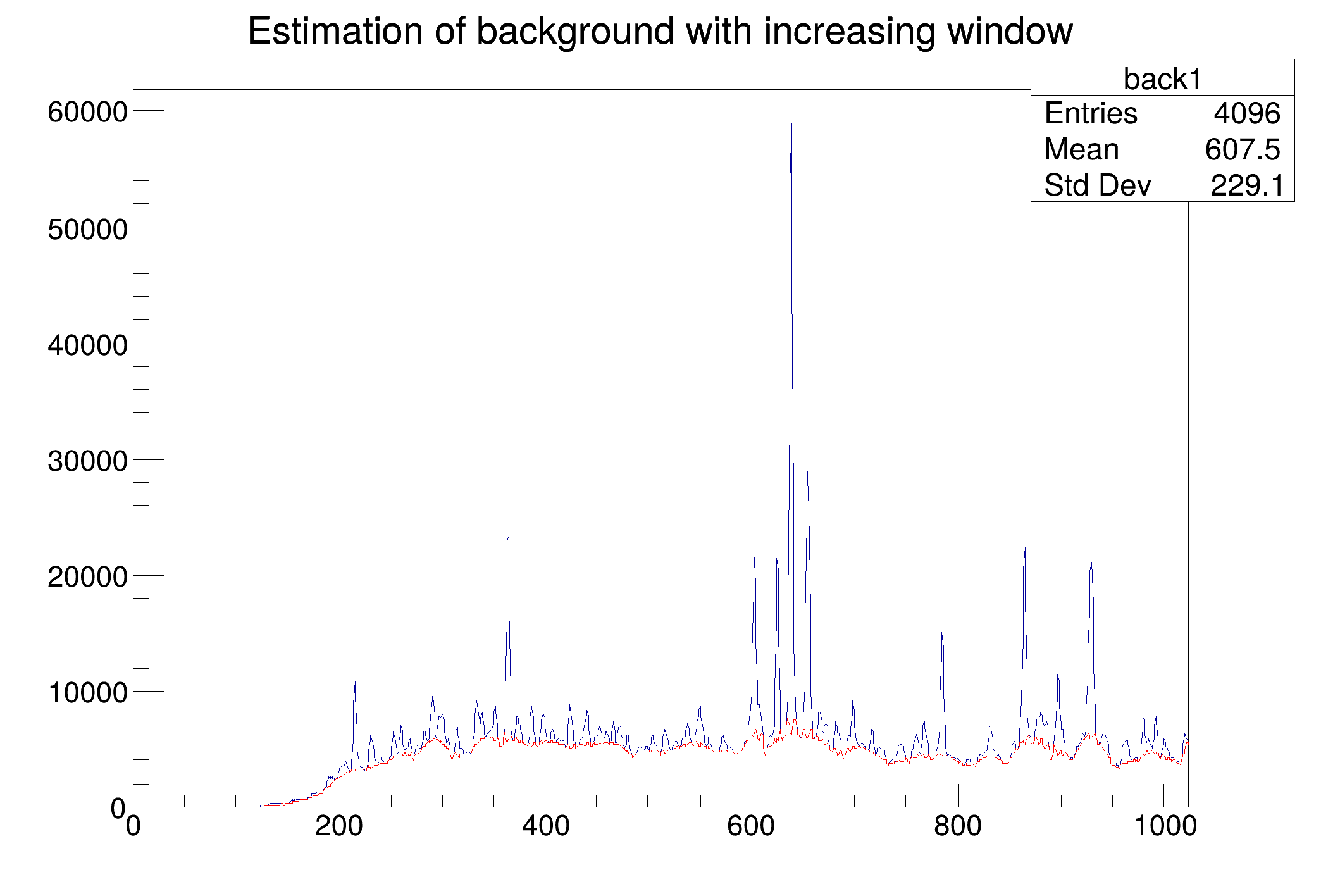### Example 2 script Background_decr.C:

In Example 1. one can notice that at the edges of the peaks the estimated background goes under the peaks. An alternative approach is to decrease the clipping window from a given value numberIterations to the value of one, which is presented in this example.

Example of the estimation of background for numberIterations=6 using decreasing clipping window algorithm. Original spectrum is shown in black color, estimated background in red color.

void Background_decr() {
Int_t i;
const Int_t nbins = 1024;
Double_t xmax = nbins;
Double_t source[nbins];
gROOT->ForceStyle();
TH1F *d = new TH1F("d","",nbins,xmin,xmax);
TString dir = gROOT->GetTutorialDir();
TString file = dir+"/spectrum/TSpectrum.root";
TFile *f = new TFile(file.Data());
TH1F *back = (TH1F*) f->Get("back1");
back->SetTitle("Estimation of background with decreasing window");
back->GetXaxis()->SetRange(1,nbins);
back->Draw("L");
TSpectrum *s = new TSpectrum();
for (i = 0; i < nbins; i++) source[i]=back->GetBinContent(i + 1);
// Estimate the background
s->Background(source,nbins,6,TSpectrum::kBackDecreasingWindow,
// Draw the estimated background
for (i = 0; i < nbins; i++) d->SetBinContent(i + 1,source[i]);
d->SetLineColor(kRed);
d->Draw("SAME L");
}
@ kBackDecreasingWindow
Definition: TSpectrum.h:42### Example 3 script Background_width.C:

The question is how to choose the width of the clipping window, i.e., numberIterations parameter. The influence of this parameter on the estimated background is illustrated in Example 3.

Example of the influence of clipping window width on the estimated background for numberIterations=4 (red line), 6 (orange line) 8 (green line) using decreasing clipping window algorithm.

in general one should set this parameter so that the value 2*numberIterations+1 was greater than the widths of preserved objects (peaks).

void Background_width() {
Int_t i;
const Int_t nbins = 1024;
Double_t xmax = nbins;
Double_t source[nbins];
gROOT->ForceStyle();
TString dir = gROOT->GetTutorialDir();
TString file = dir+"/spectrum/TSpectrum.root";
TFile *f = new TFile(file.Data());
TH1F *back = (TH1F*) f->Get("back1");
TH1F *d1 = new TH1F("d1","",nbins,xmin,xmax);
TH1F *d2 = new TH1F("d2","",nbins,xmin,xmax);
TH1F *d3 = new TH1F("d3","",nbins,xmin,xmax);
back->GetXaxis()->SetRange(1,nbins);
back->SetTitle("Influence of clipping window width on the estimated background");
back->Draw("L");
TSpectrum *s = new TSpectrum();
for (i = 0; i < nbins; i++) source[i]=back->GetBinContent(i + 1);
s->Background(source,nbins,4,TSpectrum::kBackDecreasingWindow,
for (i = 0; i < nbins; i++) d1->SetBinContent(i + 1,source[i]);
d1->Draw("SAME L");
for (i = 0; i < nbins; i++) source[i]=back->GetBinContent(i + 1);
s->Background(source,nbins,6,TSpectrum::kBackDecreasingWindow,
for (i = 0; i < nbins; i++) d2->SetBinContent(i + 1,source[i]);
d2->Draw("SAME L");
for (i = 0; i < nbins; i++) source[i]=back->GetBinContent(i + 1);
s->Background(source,nbins,8,TSpectrum::kBackDecreasingWindow,
for (i = 0; i < nbins; i++) d3->SetBinContent(i + 1,source[i]);
d3->Draw("SAME L");
}
@ kOrange
Definition: Rtypes.h:67
@ kGreen
Definition: Rtypes.h:66
virtual void SetLineColor(Color_t lcolor)
Set the line color.
Definition: TAttLine.h:40
virtual void SetBinContent(Int_t bin, Double_t content)
Set bin content see convention for numbering bins in TH1::GetBin In case the bin number is greater th...
Definition: TH1.cxx:9099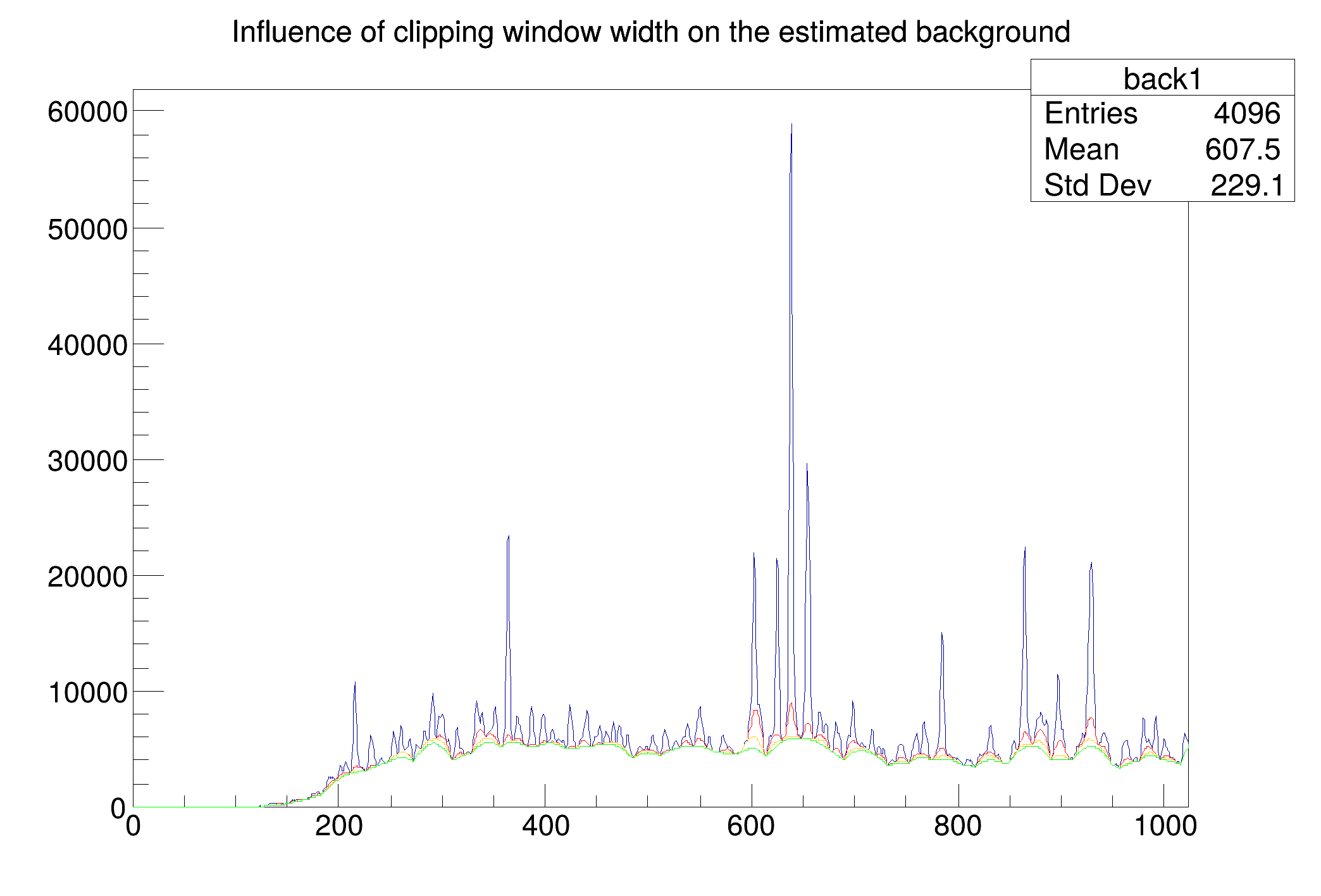### Example 4 script Background_width2.C:

another example for very complex spectrum is given here.

Example of the influence of clipping window width on the estimated background for numberIterations=10 (red line), 20 (blue line), 30 (green line) and 40 (magenta line) using decreasing clipping window algorithm.

void Background_width2() {
Int_t i;
const Int_t nbins = 4096;
Double_t xmax = 4096;
Double_t source[nbins];
gROOT->ForceStyle();
TH1F *d1 = new TH1F("d1","",nbins,xmin,xmax);
TH1F *d2 = new TH1F("d2","",nbins,xmin,xmax);
TH1F *d3 = new TH1F("d3","",nbins,xmin,xmax);
TH1F *d4 = new TH1F("d4","",nbins,xmin,xmax);
TString dir = gROOT->GetTutorialDir();
TString file = dir+"/spectrum/TSpectrum.root";
TFile *f = new TFile(file.Data());
TH1F *back = (TH1F*) f->Get("back2");
back->SetTitle("Influence of clipping window width on the estimated background");
back->SetAxisRange(0,1000);
back->SetMaximum(7000);
back->Draw("L");
TSpectrum *s = new TSpectrum();
for (i = 0; i < nbins; i++) source[i]=back->GetBinContent(i + 1);
s->Background(source,nbins,10,TSpectrum::kBackDecreasingWindow,
for (i = 0; i < nbins; i++) d1->SetBinContent(i + 1,source[i]);
d1->Draw("SAME L");
for (i = 0; i < nbins; i++) source[i]=back->GetBinContent(i + 1);
s->Background(source,nbins,20,TSpectrum::kBackDecreasingWindow,
for (i = 0; i < nbins; i++) d2->SetBinContent(i + 1,source[i]);
d2->Draw("SAME L");
for (i = 0; i < nbins; i++) source[i]=back->GetBinContent(i + 1);
s->Background(source,nbins,30,TSpectrum::kBackDecreasingWindow,
for (i = 0; i < nbins; i++) d3->SetBinContent(i + 1,source[i]);
d3->Draw("SAME L");
for (i = 0; i < nbins; i++) source[i]=back->GetBinContent(i + 1);
s->Background(source,nbins,40,TSpectrum::kBackDecreasingWindow,
for (i = 0; i < nbins; i++) d4->SetBinContent(i + 1,source[i]);
d4->Draw("SAME L");
}
@ kMagenta
Definition: Rtypes.h:66
@ kBlue
Definition: Rtypes.h:66
virtual void SetMaximum(Double_t maximum=-1111)
Definition: TH1.h:397
virtual void SetAxisRange(Double_t xmin, Double_t xmax, Option_t *axis="X")
Set the "axis" range.
Definition: Haxis.cxx:201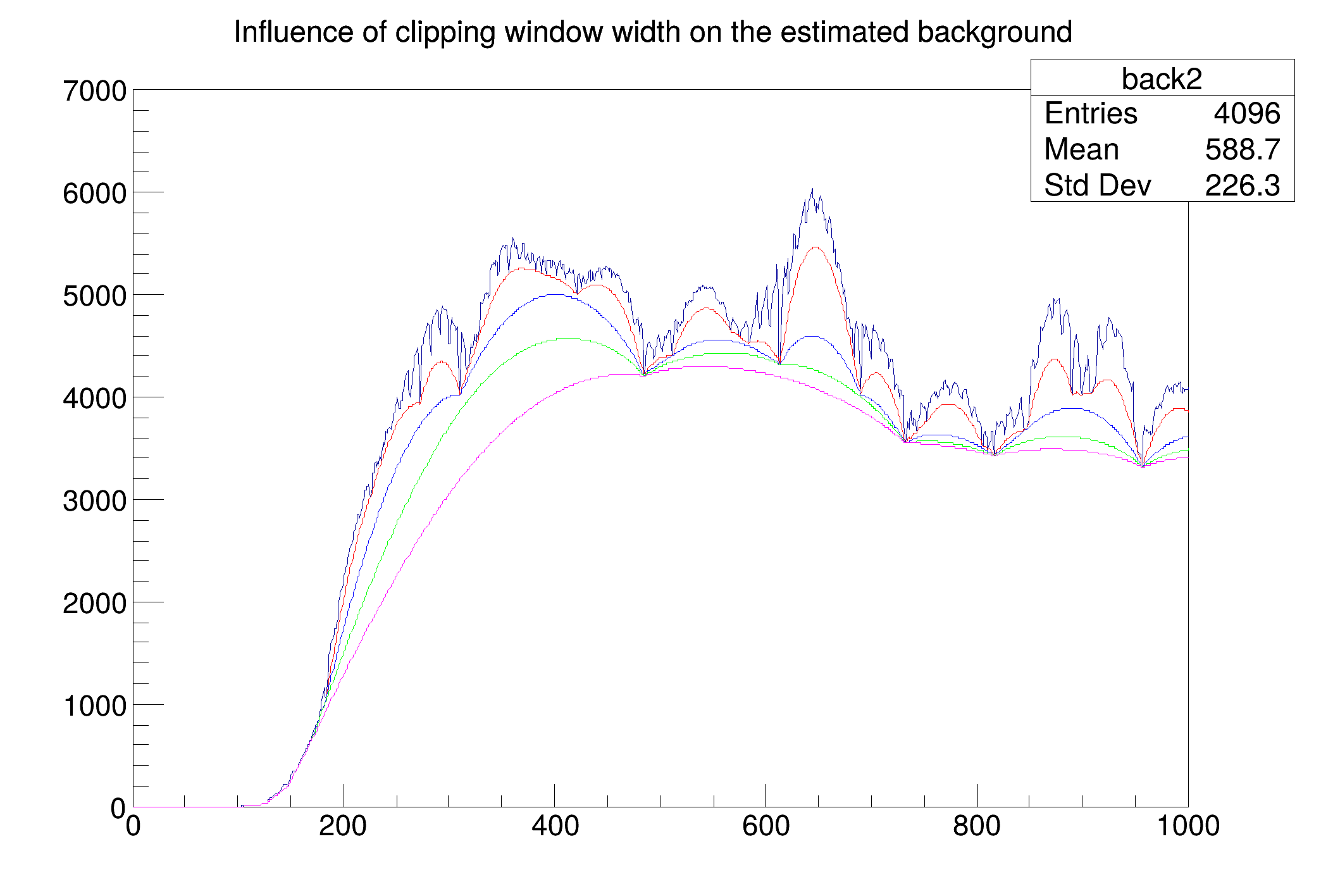### Example 5 script Background_order.C:

Second order difference filter removes linear (quasi-linear) background and preserves symmetrical peaks. However if the shape of the background is more complex one can employ higher-order clipping filters.

Example of the influence of clipping filter difference order on the estimated background for fNnumberIterations=40, 2-nd order red line, 4-th order blue line, 6-th order green line and 8-th order magenta line, and using decreasing clipping window algorithm.

void Background_order() {
Int_t i;
const Int_t nbins = 4096;
Double_t xmax = 4096;
Double_t source[nbins];
gROOT->ForceStyle();
TH1F *d1 = new TH1F("d1","",nbins,xmin,xmax);
TH1F *d2 = new TH1F("d2","",nbins,xmin,xmax);
TH1F *d3 = new TH1F("d3","",nbins,xmin,xmax);
TH1F *d4 = new TH1F("d4","",nbins,xmin,xmax);
TString dir = gROOT->GetTutorialDir();
TString file = dir+"/spectrum/TSpectrum.root";
TFile *f = new TFile(file.Data());
TH1F *back = (TH1F*) f->Get("back2");
back->SetTitle("Influence of clipping filter difference order on the estimated background");
back->SetAxisRange(1220,1460);
back->SetMaximum(3000);
back->Draw("L");
TSpectrum *s = new TSpectrum();
for (i = 0; i < nbins; i++) source[i]=back->GetBinContent(i + 1);
s->Background(source,nbins,40,TSpectrum::kBackDecreasingWindow,
for (i = 0; i < nbins; i++) d1->SetBinContent(i + 1,source[i]);
d1->Draw("SAME L");
for (i = 0; i < nbins; i++) source[i]=back->GetBinContent(i + 1);
s->Background(source,nbins,40,TSpectrum::kBackDecreasingWindow,
for (i = 0; i < nbins; i++) d2->SetBinContent(i + 1,source[i]);
d2->Draw("SAME L");
for (i = 0; i < nbins; i++) source[i]=back->GetBinContent(i + 1);
s->Background(source,nbins,40,TSpectrum::kBackDecreasingWindow,
for (i = 0; i < nbins; i++) d3->SetBinContent(i + 1,source[i]);
d3->Draw("SAME L");
for (i = 0; i < nbins; i++) source[i]=back->GetBinContent(i + 1);
s->Background(source,nbins,40,TSpectrum::kBackDecreasingWindow,
for (i = 0; i < nbins; i++) d4->SetBinContent(i + 1,source[i]);
d4->Draw("SAME L");
}
@ kBackOrder8
Definition: TSpectrum.h:40
@ kBackOrder6
Definition: TSpectrum.h:39
@ kBackOrder4
Definition: TSpectrum.h:38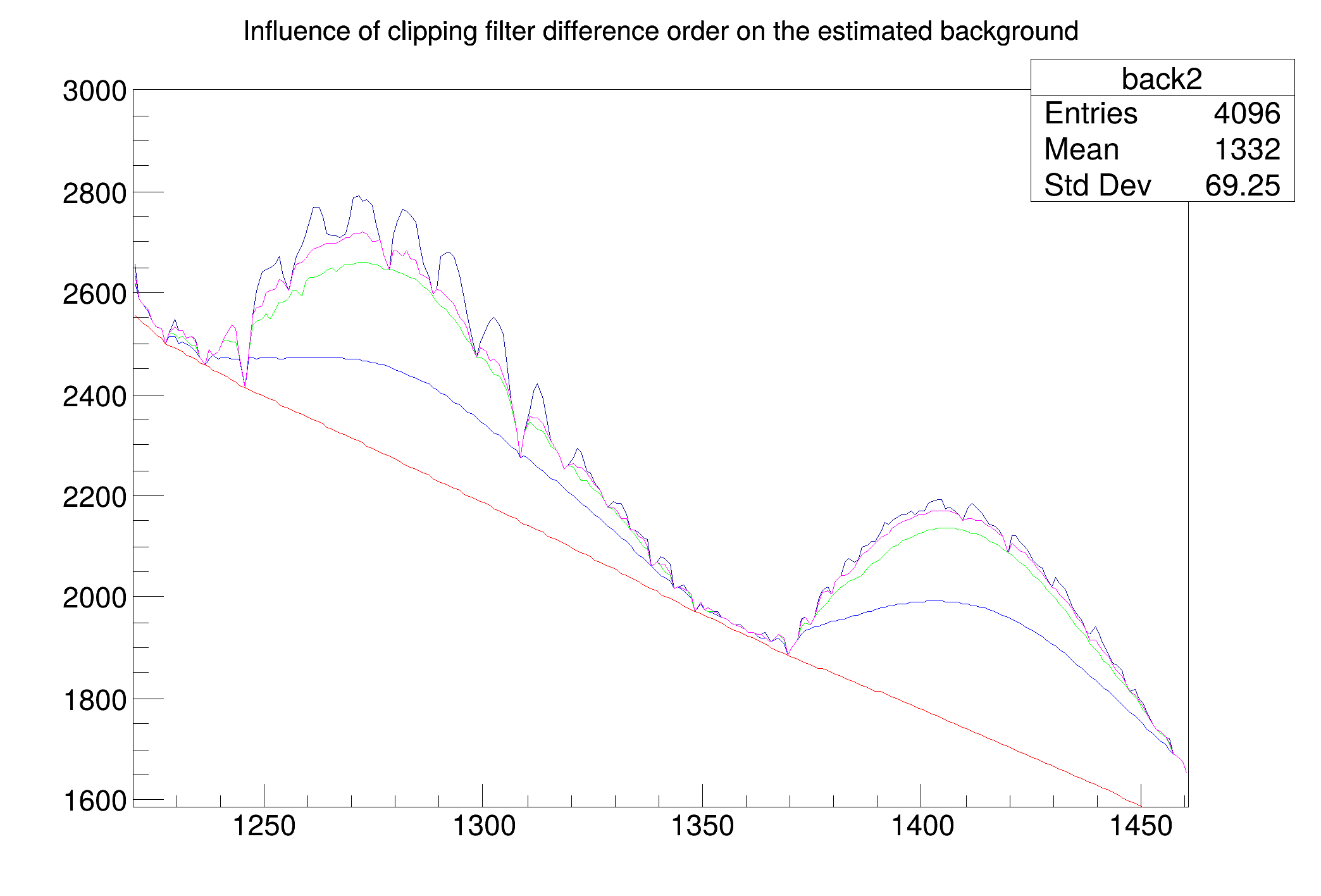### Example 6 script Background_smooth.C:

The estimate of the background can be influenced by noise present in the spectrum. We proposed the algorithm of the background estimate with simultaneous smoothing. In the original algorithm without smoothing, the estimated background snatches the lower spikes in the noise. Consequently, the areas of peaks are biased by this error.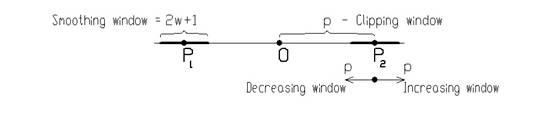Principle of background estimation algorithm with simultaneous smoothing.
void Background_smooth() {
Int_t i;
const Int_t nbins = 4096;
Double_t xmax = nbins;
Double_t source[nbins];
gROOT->ForceStyle();
TH1F *d1 = new TH1F("d1","",nbins,xmin,xmax);
TH1F *d2 = new TH1F("d2","",nbins,xmin,xmax);
TString dir = gROOT->GetTutorialDir();
TString file = dir+"/spectrum/TSpectrum.root";
TFile *f = new TFile(file.Data());
TH1F *back = (TH1F*) f->Get("back1");
back->SetTitle("Estimation of background with noise");
back->SetAxisRange(3460,3830);
back->Draw("L");
TSpectrum *s = new TSpectrum();
for (i = 0; i < nbins; i++) source[i]=back->GetBinContent(i + 1);
s->Background(source,nbins,6,TSpectrum::kBackDecreasingWindow,
for (i = 0; i < nbins; i++) d1->SetBinContent(i + 1,source[i]);
d1->Draw("SAME L");
for (i = 0; i < nbins; i++) source[i]=back->GetBinContent(i + 1);
s->Background(source,nbins,6,TSpectrum::kBackDecreasingWindow,
for (i = 0; i < nbins; i++) d2->SetBinContent(i + 1,source[i]);
d2->Draw("SAME L");
}
const Bool_t kTRUE
Definition: RtypesCore.h:100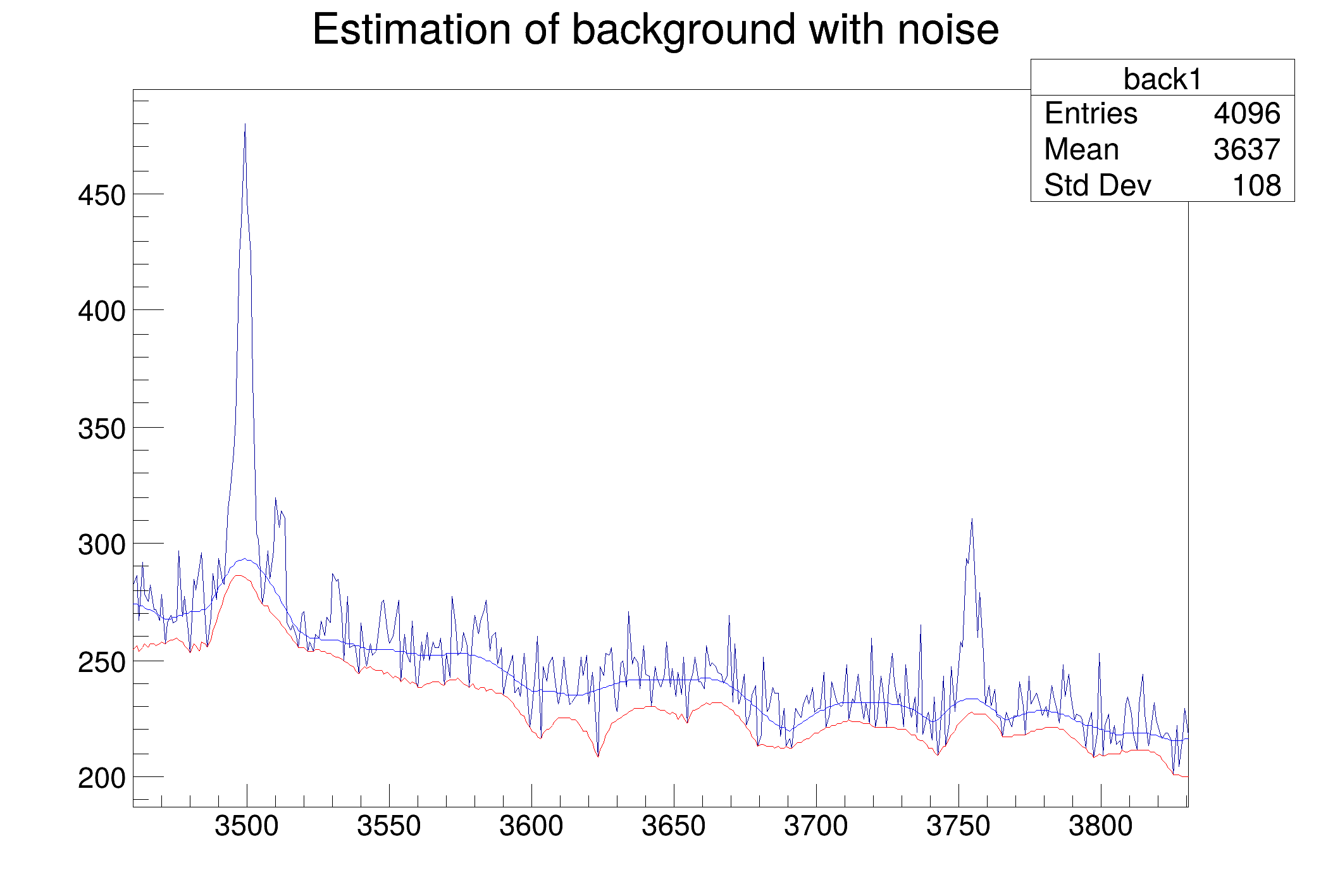### Example 8 script Background_compton.C:

Sometimes it is necessary to include also the Compton edges into the estimate of the background. This example presents the synthetic spectrum with Compton edges. The background was estimated using the 8-th order filter with the estimation of the Compton edges using decreasing clipping window algorithm (numberIterations=10) with smoothing (smoothingWindow=5).

Example of the estimate of the background with Compton edges (red line) for numberIterations=10, 8-th order difference filter, using decreasing clipping window algorithm and smoothing (smoothingWindow=5).

void Background_compton() {
Int_t i;
const Int_t nbins = 256;
Double_t xmax = nbins;
Double_t source[nbins];
gROOT->ForceStyle();
TH1F *d1 = new TH1F("d1","",nbins,xmin,xmax);
TString dir = gROOT->GetTutorialDir();
TString file = dir+"/spectrum/TSpectrum.root";
TFile *f = new TFile(file.Data());
TH1F *back = (TH1F*) f->Get("back3");
back->SetTitle("Estimation of background with Compton edges under peaks");
back->GetXaxis()->SetRange(1,nbins);
back->Draw("L");
TSpectrum *s = new TSpectrum();
for (i = 0; i < nbins; i++) source[i]=back->GetBinContent(i + 1);
s->Background(source,nbins,10,TSpectrum::kBackDecreasingWindow,
for (i = 0; i < nbins; i++) d1->SetBinContent(i + 1,source[i]);
d1->Draw("SAME L");
}
@ kBackSmoothing5
Definition: TSpectrum.h:44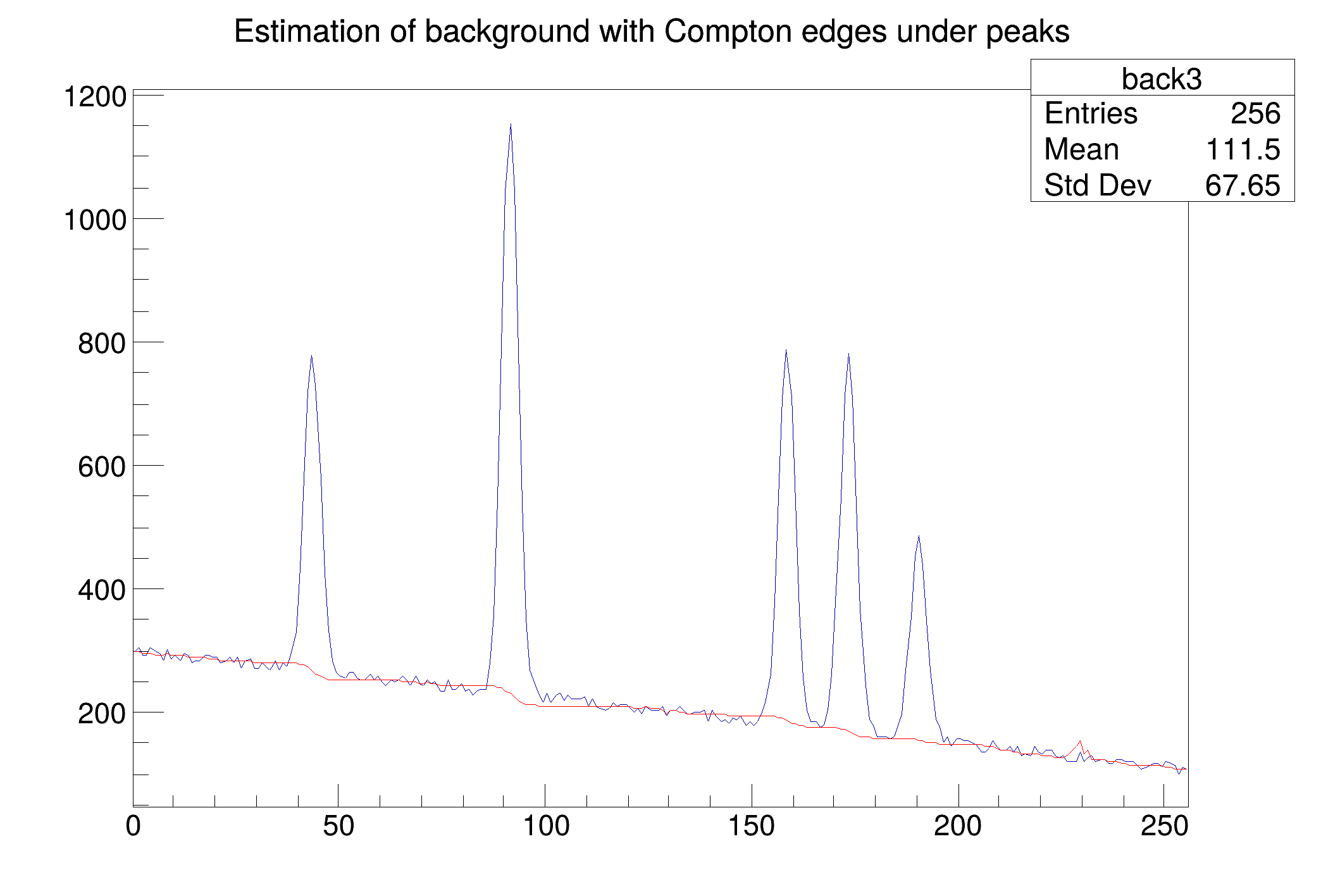Definition at line 515 of file TSpectrum.cxx.

## ◆ Class()

 static TClass * TSpectrum::Class ( )
static
Returns
TClass describing this class

## ◆ Class_Name()

 static const char * TSpectrum::Class_Name ( )
static
Returns
Name of this class

## ◆ Class_Version()

 static Version_t TSpectrum::Class_Version ( )
inlinestatic
Returns
Version of this class

Definition at line 78 of file TSpectrum.h.

## ◆ DeclFileName()

 static const char * TSpectrum::DeclFileName ( )
inlinestatic
Returns
Name of the file containing the class declaration

Definition at line 78 of file TSpectrum.h.

## ◆ Deconvolution()

 const char * TSpectrum::Deconvolution ( Double_t * source, const Double_t * response, Int_t ssize, Int_t numberIterations, Int_t numberRepetitions, Double_t boost )

One-dimensional deconvolution function.

This function calculates deconvolution from source spectrum according to response spectrum using Gold deconvolution algorithm. The result is placed in the vector pointed by source pointer. On successful completion it returns 0. On error it returns pointer to the string describing error. If desired after every numberIterations one can apply boosting operation (exponential function with exponent given by boost coefficient) and repeat it numberRepetitions times.

#### Parameters:

• source: pointer to the vector of source spectrum
• response: pointer to the vector of response spectrum
• ssize: length of source and response spectra
• numberIterations, for details we refer to the reference given below
• numberRepetitions, for repeated boosted deconvolution
• boost, boosting coefficient

The goal of this function is the improvement of the resolution in spectra, decomposition of multiplets. The mathematical formulation of the convolution system is:

$y(i) = \sum_{k=0}^{N-1} h(i-k)x(k), i=0,1,2,...,N-1$

where h(i) is the impulse response function, x, y are input and output vectors, respectively, N is the length of x and h vectors. In matrix form we have:

$\begin{bmatrix} y(0) \\ y(1) \\ \dots \\ y(2N-2) \\ y(2N-1) \end{bmatrix} = \begin{bmatrix} h(0) & 0 & 0 & \dots & 0 \\ h(1) & h(0) & 0 & \dots & \dots \\ \dots & h(1) & h(0) & \dots & \dots \\ \dots & \dots & h(1) & \dots & \dots \\ \dots & \dots & \dots & \dots & \dots \\ h(N-1) & \dots & \dots &\dots & 0 \\ 0 & h(N-1) & \dots & \dots & h(0) \\ 0 & 0 & h(N-1) & \dots & h(1) \\ \dots & \dots & \dots & \dots & \dots \\ 0 & 0 & 0 & \dots & h(N-1) \end{bmatrix} \begin{bmatrix} x(0) \\ x(1) \\ \dots \\ x(N-1) \end{bmatrix}$

Let us assume that we know the response and the output vector (spectrum) of the above given system. The deconvolution represents solution of the overdetermined system of linear equations, i.e., the calculation of the vector x. From numerical stability point of view the operation of deconvolution is extremely critical (ill-posed problem) as well as time consuming operation. The Gold deconvolution algorithm proves to work very well, other methods (Fourier, VanCittert etc) oscillate. It is suitable to process positive definite data (e.g. histograms).

#### Gold deconvolution algorithm:

$y = Hx \\ H^{T}y = H^{T}Hx \\ y^{'} = H^{'}x \\ x_{i}^{(k+1)} = \frac{y_{i}^{'}}{\sum_{m=0}^{N-1}H_{im}^{'}x_{m}{(k)}}x_{i}{(k)}, i=0,1,2,...,N-1 \\ k = 1,2,3,...,L x^{0} = [1,1, ..., 1]^T$

Where L is given number of iterations (numberIterations parameter).

#### Boosted deconvolution:

1. Set the initial solution: $$x^{(0)} = [1,1,...,1]^{T}$$
2. Set required number of repetitions R and iterations L.
3. Set r = 1.
4. Using Gold deconvolution algorithm for k=1,2,...,L find $$x^{(L)}$$
5. If r = R stop calculation, else
1. Apply boosting operation, i.e., set $$x^{(0)}(i) = [x^{(L)}(i)]^{p}$$ i=0,1,...N-1 and p is boosting coefficient >0.
2. r = r + 1
3. continue in 4.

#### References:

1. Gold R., ANL-6984, Argonne National Laboratories, Argonne Ill, 1964.
2. Coote G.E., Iterative smoothing and deconvolution of one- and two-dimensional elemental distribution data, NIM B 130 (1997) 118.
3. M. Morhac;, J. Kliman, V. Matouoek, M. Veselsky, I. Turzo: Efficient one- and two-dimensional Gold deconvolution and its application to gamma-ray spectra decomposition. NIM, A401 (1997) 385-408.
4. Morhac; M., Matouoek V., Kliman J., Efficient algorithm of multidimensional deconvolution and its application to nuclear data processing, Digital Signal Processing 13 (2003) 144.

### Example 8 - script Deconvolution.C :

response function (usually peak) should be shifted left to the first non-zero channel (bin).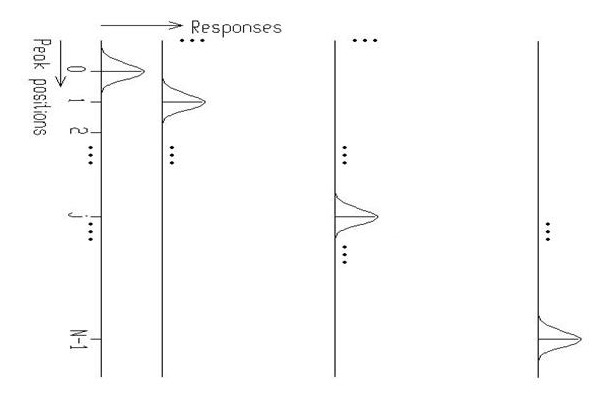Principle how the response matrix is composed inside of the Deconvolution function.
void Deconvolution() {
Int_t i;
const Int_t nbins = 256;
Double_t xmax = nbins;
Double_t source[nbins];
Double_t response[nbins];
gROOT->ForceStyle();
TString dir = gROOT->GetTutorialDir();
TString file = dir+"/spectrum/TSpectrum.root";
TFile *f = new TFile(file.Data());
TH1F *h = (TH1F*) f->Get("decon1");
h->SetTitle("Deconvolution");
TH1F *d = (TH1F*) f->Get("decon_response");
for (i = 0; i < nbins; i++) source[i]=h->GetBinContent(i + 1);
for (i = 0; i < nbins; i++) response[i]=d->GetBinContent(i + 1);
h->SetMaximum(30000);
h->Draw("L");
TSpectrum *s = new TSpectrum();
s->Deconvolution(source,response,256,1000,1,1);
for (i = 0; i < nbins; i++) d->SetBinContent(i + 1,source[i]);
d->SetLineColor(kRed);
d->Draw("SAME L");
}
#define h(i)
Definition: RSha256.hxx:106
const char * Deconvolution(Double_t *source, const Double_t *response, Int_t ssize, Int_t numberIterations, Int_t numberRepetitions, Double_t boost)
One-dimensional deconvolution function.
Definition: TSpectrum.cxx:1462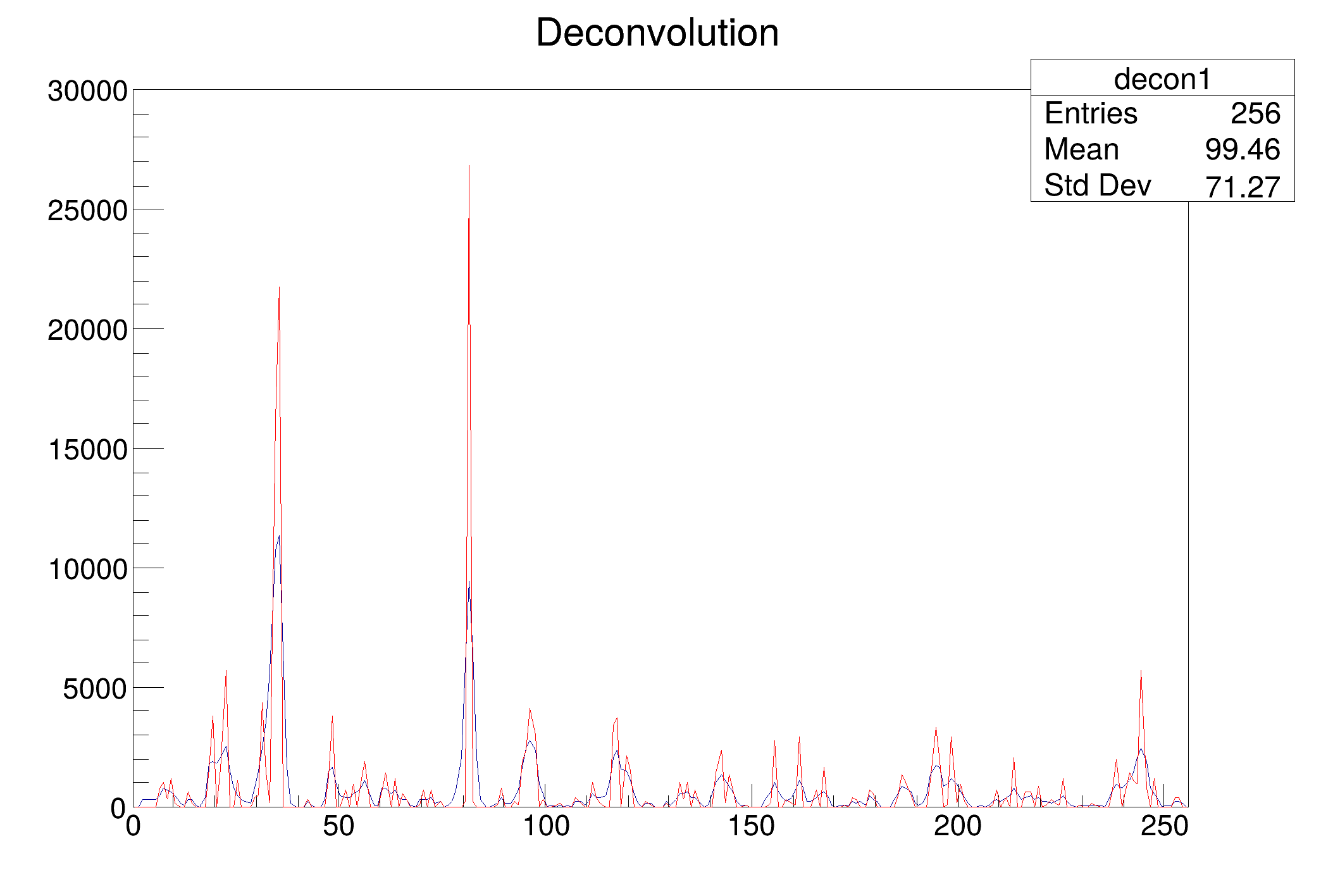### Examples of Gold deconvolution method:

First let us study the influence of the number of iterations on the deconvolved spectrum (Fig. 12).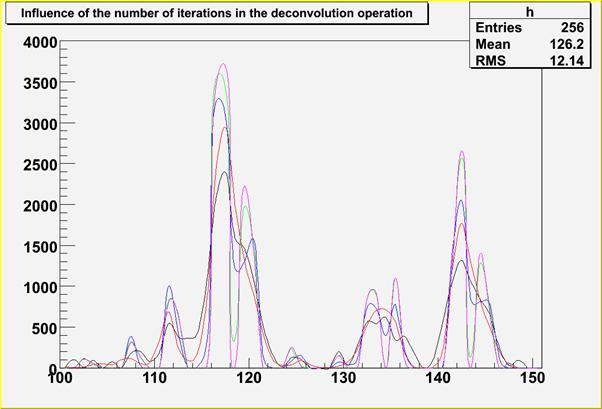Fig. 12 Study of Gold deconvolution algorithm.The original source spectrum is drawn with black color, spectrum after 100 iterations with red color, spectrum after 1000 iterations with blue color, spectrum after 10000 iterations with green color and spectrum after 100000 iterations with magenta color.

For relatively narrow peaks in the above given example the Gold deconvolution method is able to decompose overlapping peaks practically to delta - functions. In the next example we have chosen a synthetic data (spectrum, 256 channels) consisting of 5 very closely positioned, relatively wide peaks (sigma =5), with added noise (Fig. 13). Thin lines represent pure Gaussians (see Table 1); thick line is a resulting spectrum with additive noise (10% of the amplitude of small peaks).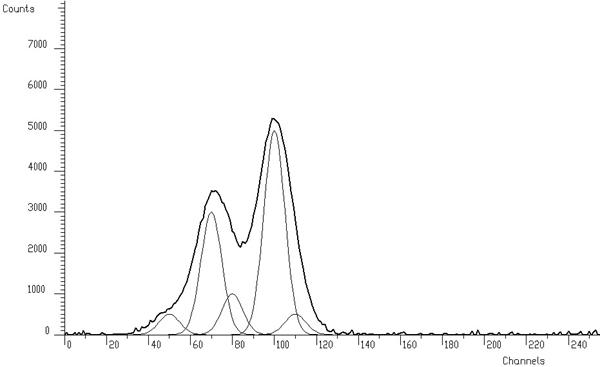Fig. 13 Testing example of synthetic spectrum composed of 5 Gaussians with added noise.
Peak # Position Height Area
1 50 500 10159
2 70 3000 60957
3 80 1000 20319
4 100 5000 101596
5 110 500 10159

Table 1 Positions, heights and areas of peaks in the spectrum shown in Fig. 13.

In ideal case, we should obtain the result given in Fig. 14. The areas of the Gaussian components of the spectrum are concentrated completely to delta-functions. When solving the overdetermined system of linear equations with data from Fig. 13 in the sense of minimum least squares criterion without any regularisation we obtain the result with large oscillations (Fig. 15). From mathematical point of view, it is the optimal solution in the unconstrained space of independent variables. From physical point of view we are interested only in a meaningful solution. Therefore, we have to employ regularisation techniques (e.g. Gold deconvolution) and/or to confine the space of allowed solutions to subspace of positive solutions.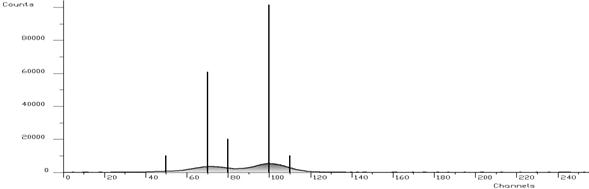Fig. 14 The same spectrum like in Fig. 13, outlined bars show the contents of present components (peaks).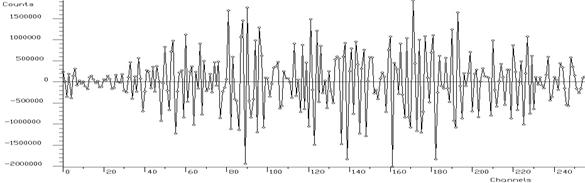Fig. 15 Least squares solution of the system of linear equations without regularisation.

### Example 9 - script Deconvolution_wide.C

When we employ Gold deconvolution algorithm we obtain the result given in Fig. 16. One can observe that the resulting spectrum is smooth. On the other hand the method is not able to decompose completely the peaks in the spectrum.

Example of Gold deconvolution for closely positioned wide peaks. The original source spectrum is drawn with black color, the spectrum after the deconvolution (10000 iterations) with red color.

void Deconvolution_wide() {
Int_t i;
const Int_t nbins = 256;
Double_t xmax = nbins;
Double_t source[nbins];
Double_t response[nbins];
gROOT->ForceStyle();
TString dir = gROOT->GetTutorialDir();
TString file = dir+"/spectrum/TSpectrum.root";
TFile *f = new TFile(file.Data());
TH1F *h = (TH1F*) f->Get("decon3");
h->SetTitle("Deconvolution of closely positioned overlapping peaks using Gold deconvolution method");
TH1F *d = (TH1F*) f->Get("decon_response_wide");
for (i = 0; i < nbins; i++) source[i] = h->GetBinContent(i + 1);
for (i = 0; i < nbins; i++) response[i] = d->GetBinContent(i + 1);
h->SetMaximum(50000);
h->Draw("L");
TSpectrum *s = new TSpectrum();
s->Deconvolution(source,response,256,10000,1,1);
for (i = 0; i < nbins; i++) d->SetBinContent(i + 1,source[i]);
d->SetLineColor(kRed);
d->Draw("SAME L");
}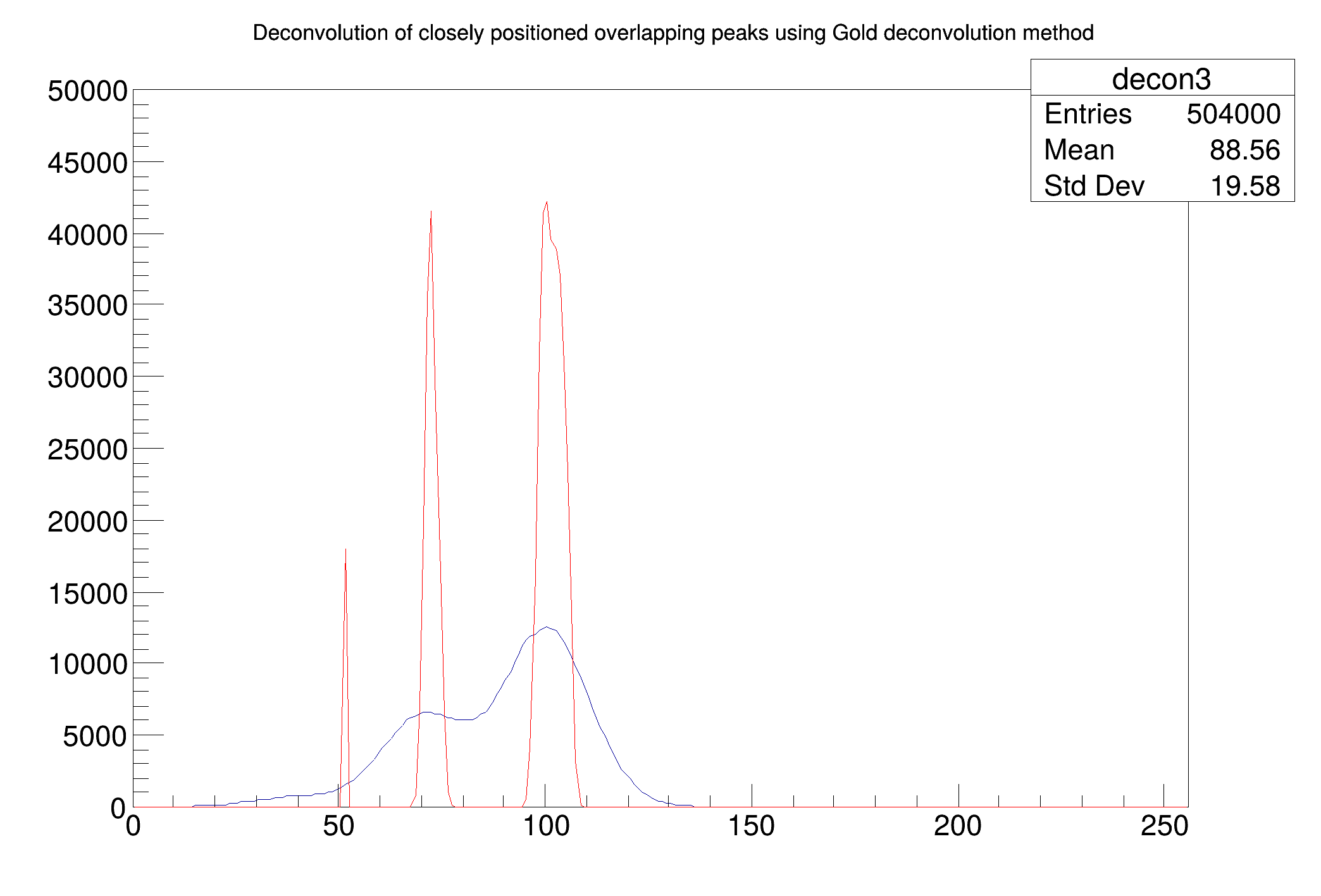### Example 10 - script Deconvolution_wide_boost.C :

Further let us employ boosting operation into deconvolution (Fig. 17).

The original source spectrum is drawn with black color, the spectrum after the deconvolution with red color. Number of iterations = 200, number of repetitions = 50 and boosting coefficient = 1.2.

One can observe that peaks are decomposed practically to delta functions. Number of peaks is correct, positions of big peaks as well as their areas are relatively well estimated. However there is a considerable error in the estimation of the position of small right hand peak.

void Deconvolution_wide_boost() {
Int_t i;
const Int_t nbins = 256;
Double_t xmax = nbins;
Double_t source[nbins];
Double_t response[nbins];
gROOT->ForceStyle();
TH1F *h = new TH1F("h","Deconvolution",nbins,xmin,xmax);
TH1F *d = new TH1F("d","",nbins,xmin,xmax);
TString dir = gROOT->GetTutorialDir();
TString file = dir+"/spectrum/TSpectrum.root";
TFile *f = new TFile(file.Data());
h = (TH1F*) f->Get("decon3");
h->SetTitle("Deconvolution of closely positioned overlapping peaks using boosted Gold deconvolution method");
d = (TH1F*) f->Get("decon_response_wide");
for (i = 0; i < nbins; i++) source[i]=h->GetBinContent(i + 1);
for (i = 0; i < nbins; i++) response[i]=d->GetBinContent(i + 1);
h->SetMaximum(200000);
h->Draw("L");
TSpectrum *s = new TSpectrum();
s->Deconvolution(source,response,256,200,50,1.2);
for (i = 0; i < nbins; i++) d->SetBinContent(i + 1,source[i]);
d->SetLineColor(kRed);
d->Draw("SAME L");
}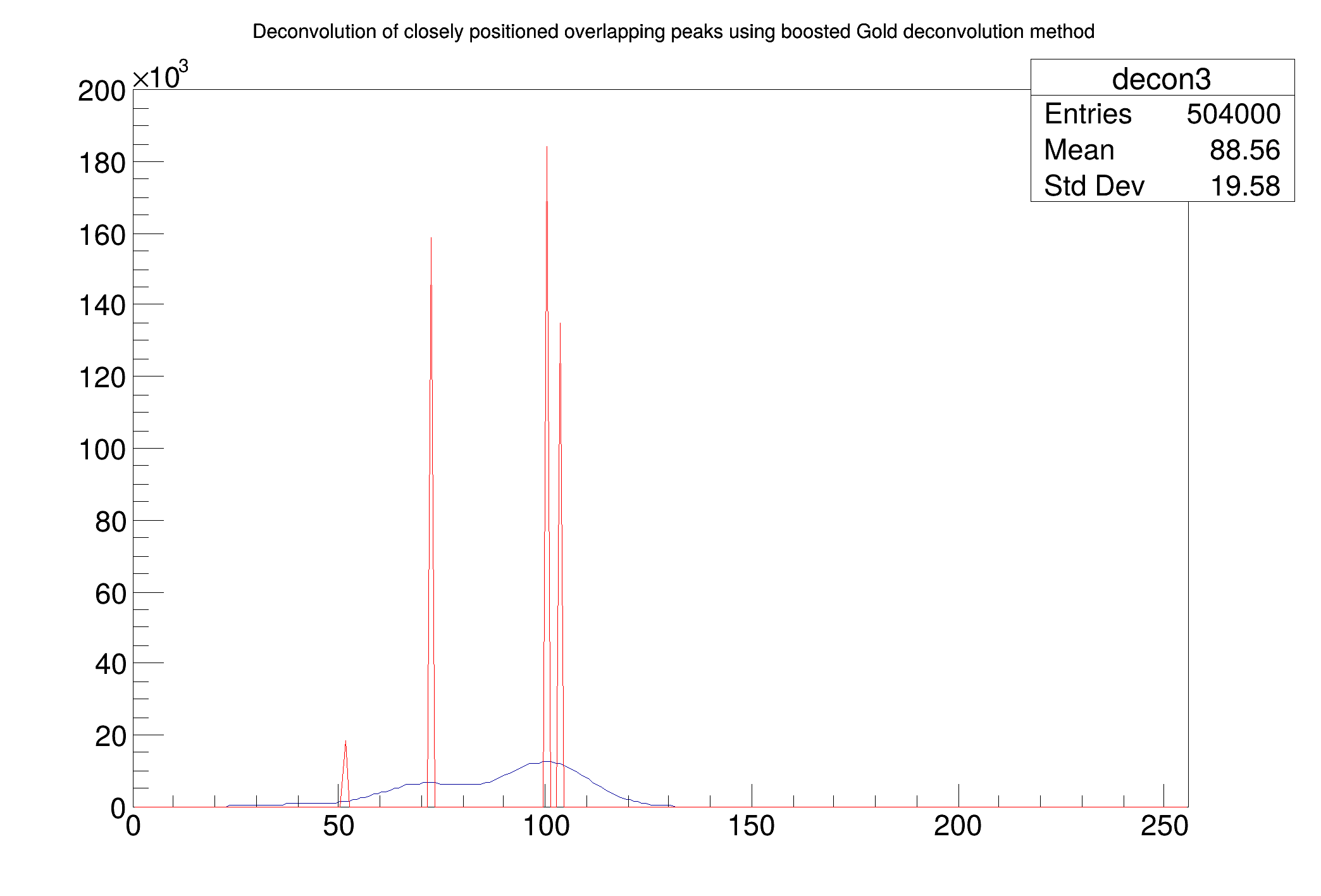Definition at line 1462 of file TSpectrum.cxx.

## ◆ DeconvolutionRL()

 const char * TSpectrum::DeconvolutionRL ( Double_t * source, const Double_t * response, Int_t ssize, Int_t numberIterations, Int_t numberRepetitions, Double_t boost )

One-dimensional deconvolution function.

This function calculates deconvolution from source spectrum according to response spectrum using Richardson-Lucy deconvolution algorithm. The result is placed in the vector pointed by source pointer. On successful completion it returns 0. On error it returns pointer to the string describing error. If desired after every numberIterations one can apply boosting operation (exponential function with exponent given by boost coefficient) and repeat it numberRepetitions times (see Gold deconvolution).

#### Parameters:

• source: pointer to the vector of source spectrum
• response: pointer to the vector of response spectrum
• ssize: length of source and response spectra
• numberIterations, for details we refer to the reference given above
• numberRepetitions, for repeated boosted deconvolution
• boost, boosting coefficient

### Richardson-Lucy deconvolution algorithm:

For discrete systems it has the form:

$x^{(n)}(i) = x^{(n-1)}(i) \sum_{j=0}^{N-1}h(i,j)\frac{y(j)}{\sum_{k=0}^{M-1}h(j,k)x^{(n-1)}(k)} \\ i \in \left<0,M-1\right>$

for positive input data and response matrix this iterative method forces the deconvoluted spectra to be non-negative. The Richardson-Lucy iteration converges to the maximum likelihood solution for Poisson statistics in the data.

#### References:

1. Abreu M.C. et al., A four-dimensional deconvolution method to correct NA38 experimental data, NIM A 405 (1998) 139.
2. Lucy L.B., A.J. 79 (1974) 745.
3. Richardson W.H., J. Opt. Soc. Am. 62 (1972) 55.

### Example 11 - script DeconvolutionRL_wide.C :

When we employ Richardson-Lucy deconvolution algorithm to our data from Fig. 13 we obtain the following result. One can observe improvements as compared to the result achieved by Gold deconvolution. Nevertheless it is unable to decompose the multiplet.

Example of Richardson-Lucy deconvolution for closely positioned wide peaks. The original source spectrum is drawn with black color, the spectrum after the deconvolution (10000 iterations) with red color.

void DeconvolutionRL_wide() {
Int_t i;
const Int_t nbins = 256;
Double_t xmax = nbins;
Double_t source[nbins];
Double_t response[nbins];
gROOT->ForceStyle();
TString dir = gROOT->GetTutorialDir();
TString file = dir+"/spectrum/TSpectrum.root";
TFile *f = new TFile(file.Data());
TH1F* h = (TH1F*) f->Get("decon3");
h->SetTitle("Deconvolution of closely positioned overlapping peaks using Richardson-Lucy deconvolution method");
TH1F* d = (TH1F*) f->Get("decon_response_wide");
for (i = 0; i < nbins; i++) source[i]=h->GetBinContent(i + 1);
for (i = 0; i < nbins; i++) response[i]=d->GetBinContent(i + 1);
h->SetMaximum(30000);
h->Draw("L");
TSpectrum *s = new TSpectrum();
s->DeconvolutionRL(source,response,256,10000,1,1);
for (i = 0; i < nbins; i++) d->SetBinContent(i + 1,source[i]);
d->SetLineColor(kRed);
d->Draw("SAME L");
}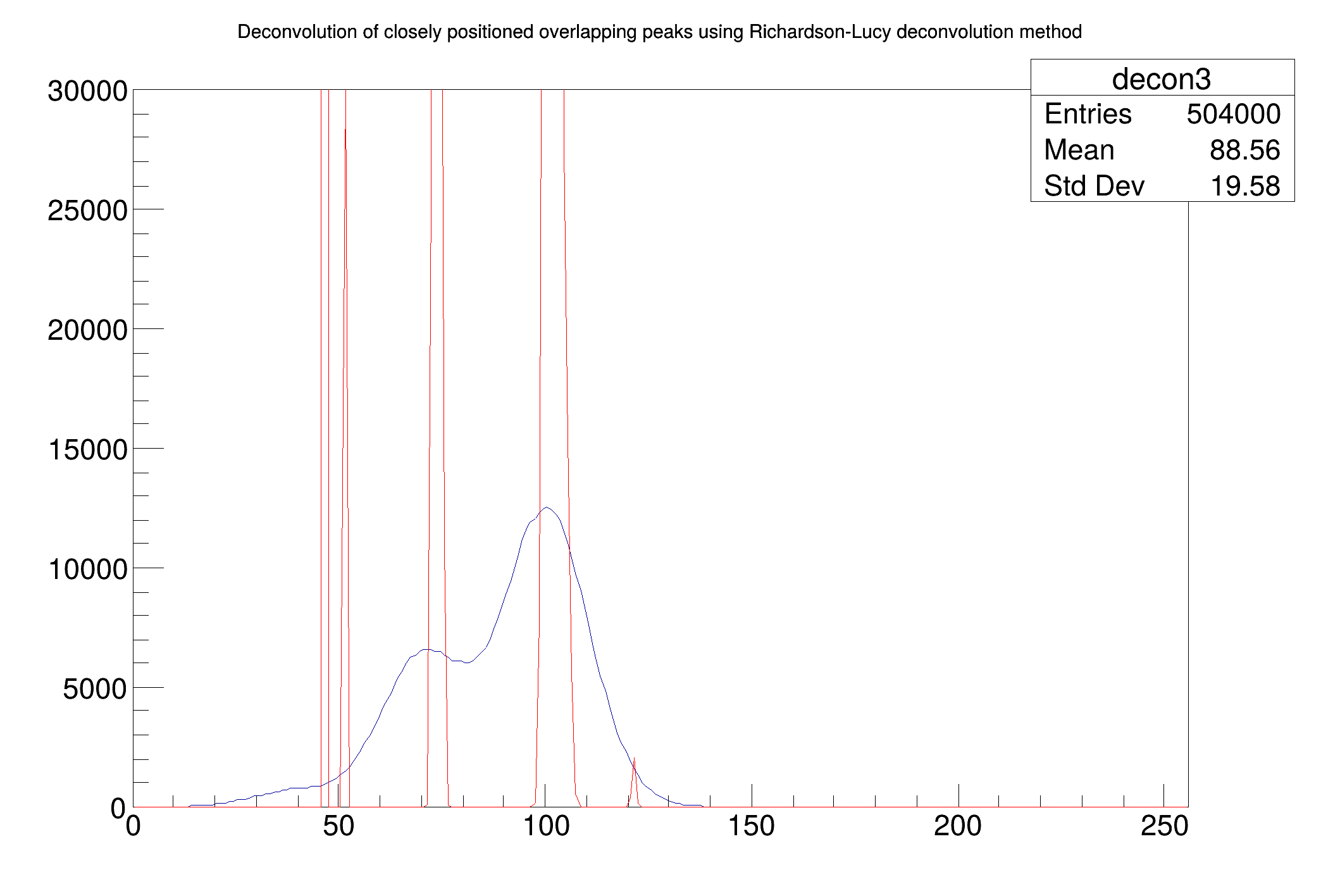### Example 12 - script DeconvolutionRL_wide_boost.C :

Further let us employ boosting operation into deconvolution.

The original source spectrum is drawn with black color, the spectrum after the deconvolution with red color. Number of iterations = 200, number of repetitions = 50 and boosting coefficient = 1.2.

One can observe improvements in the estimation of peak positions as compared to the results achieved by Gold deconvolution.

void DeconvolutionRL_wide_boost() {
Int_t i;
const Int_t nbins = 256;
Double_t xmax = nbins;
Double_t source[nbins];
Double_t response[nbins];
gROOT->ForceStyle();
TString dir = gROOT->GetTutorialDir();
TString file = dir+"/spectrum/TSpectrum.root";
TFile *f = new TFile(file.Data());
TH1F* h = (TH1F*) f->Get("decon3");
h->SetTitle("Deconvolution of closely positioned overlapping peaks using boosted Richardson-Lucy deconvolution method");
TH1F* d = (TH1F*) f->Get("decon_response_wide");
for (i = 0; i < nbins; i++) source[i]=h->GetBinContent(i + 1);
for (i = 0; i < nbins; i++) response[i]=d->GetBinContent(i + 1);
h->Draw("L");
TSpectrum *s = new TSpectrum();
s->DeconvolutionRL(source,response,256,200,50,1.2);
for (i = 0; i < nbins; i++) d->SetBinContent(i + 1,source[i]);
d->SetLineColor(kRed);
d->Draw("SAME L");
}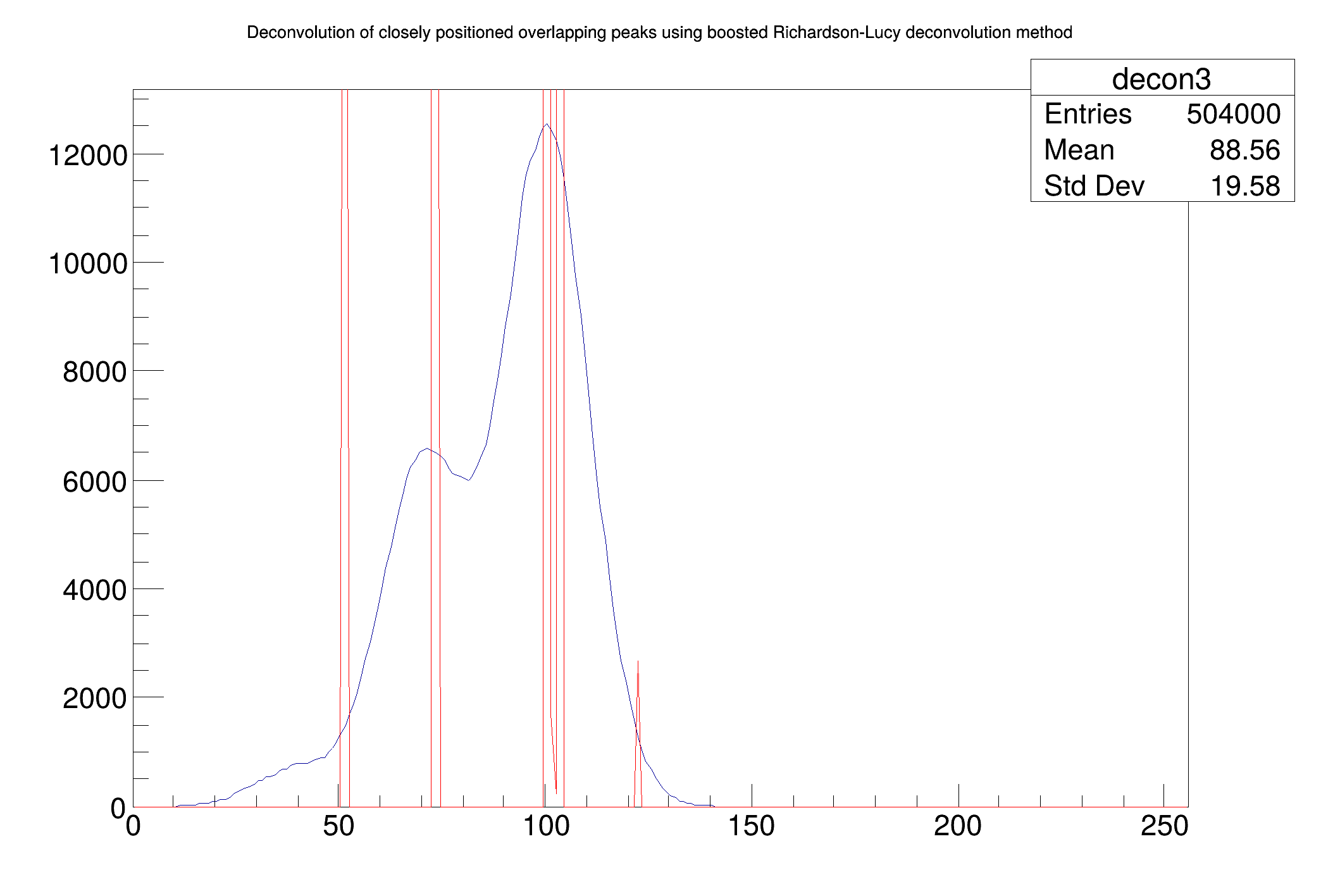Definition at line 1668 of file TSpectrum.cxx.

## ◆ GetHistogram()

 TH1 * TSpectrum::GetHistogram ( ) const
inline

Definition at line 56 of file TSpectrum.h.

## ◆ GetNPeaks()

 Int_t TSpectrum::GetNPeaks ( ) const
inline

Definition at line 57 of file TSpectrum.h.

## ◆ GetPositionX()

 Double_t * TSpectrum::GetPositionX ( ) const
inline

Definition at line 58 of file TSpectrum.h.

## ◆ GetPositionY()

 Double_t * TSpectrum::GetPositionY ( ) const
inline

Definition at line 59 of file TSpectrum.h.

## ◆ IsA()

 TClass * TSpectrum::IsA ( ) const
inlineoverridevirtual
Returns
TClass describing current object

Reimplemented from TNamed.

Definition at line 78 of file TSpectrum.h.

## ◆ operator=()

 TSpectrum & TSpectrum::operator= ( const TSpectrum & )
private

## ◆ Print()

 void TSpectrum::Print ( Option_t * option = "" ) const
overridevirtual

Print the array of positions.

Reimplemented from TNamed.

Definition at line 222 of file TSpectrum.cxx.

## ◆ Search()

 Int_t TSpectrum::Search ( const TH1 * hin, Double_t sigma = 2, Option_t * option = "", Double_t threshold = 0.05 )
virtual

One-dimensional peak search function.

This function searches for peaks in source spectrum in hin The number of found peaks and their positions are written into the members fNpeaks and fPositionX. The search is performed in the current histogram range.

#### Parameters:

• hin: pointer to the histogram of source spectrum
• sigma: sigma of searched peaks, for details we refer to manual
• threshold: (default=0.05) peaks with amplitude less than threshold*highest_peak are discarded. 0<threshold<1

By default, the background is removed before deconvolution. Specify the option "nobackground" to not remove the background.

By default the "Markov" chain algorithm is used. Specify the option "noMarkov" to disable this algorithm Note that by default the source spectrum is replaced by a new spectrum

By default a polymarker object is created and added to the list of functions of the histogram. The histogram is drawn with the specified option and the polymarker object drawn on top of the histogram. The polymarker coordinates correspond to the npeaks peaks found in the histogram.

A pointer to the polymarker object can be retrieved later via:

TList *functions = hin->GetListOfFunctions();
TPolyMarker *pm = (TPolyMarker*)functions->FindObject("TPolyMarker");
Definition: TList.h:38
TObject * FindObject(const char *name) const override
Find an object in this list using its name.
Definition: TList.cxx:578
A PolyMarker is defined by an array on N points in a 2-D space.
Definition: TPolyMarker.h:31

Specify the option "goff" to disable the storage and drawing of the polymarker.

To disable the final drawing of the histogram with the search results (in case you want to draw it yourself) specify "nodraw" in the options parameter.

Definition at line 269 of file TSpectrum.cxx.

## ◆ Search1HighRes()

 Int_t TSpectrum::Search1HighRes ( Double_t * source, Double_t * destVector, Int_t ssize, Double_t sigma, Double_t threshold, bool backgroundRemove, Int_t deconIterations, bool markov, Int_t averWindow )

Old name of SearcHighRes introduced for back compatibility.

This function will be removed after the June 2006 release

Definition at line 2566 of file TSpectrum.cxx.

## ◆ SearchHighRes()

 Int_t TSpectrum::SearchHighRes ( Double_t * source, Double_t * destVector, Int_t ssize, Double_t sigma, Double_t threshold, bool backgroundRemove, Int_t deconIterations, bool markov, Int_t averWindow )

One-dimensional high-resolution peak search function.

This function searches for peaks in source spectrum. It is based on deconvolution method. First the background is removed (if desired), then Markov smoothed spectrum is calculated (if desired), then the response function is generated according to given sigma and deconvolution is carried out. The order of peaks is arranged according to their heights in the spectrum after background elimination. The highest peak is the first in the list. On success it returns number of found peaks.

#### Parameters:

• source: pointer to the vector of source spectrum.
• destVector: pointer to the vector of resulting deconvolved spectrum.
• ssize: length of source spectrum.
• sigma: sigma of searched peaks, for details we refer to manual.
• threshold: threshold value in % for selected peaks, peaks with amplitude less than threshold*highest_peak/100 are ignored, see manual.
• backgroundRemove: logical variable, set if the removal of background before deconvolution is desired.
• deconIterations-number of iterations in deconvolution operation.
• markov: logical variable, if it is true, first the source spectrum is replaced by new spectrum calculated using Markov chains method.
• averWindow: averaging window of searched peaks, for details we refer to manual (applies only for Markov method).

### Peaks searching:

The goal of this function is to identify automatically the peaks in spectrum with the presence of the continuous background and statistical fluctuations - noise.

The common problems connected with correct peak identification are:

• non-sensitivity to noise, i.e., only statistically relevant peaks should be identified.
• non-sensitivity of the algorithm to continuous background.
• ability to identify peaks close to the edges of the spectrum region. Usually peak finders fail to detect them.
• resolution, decomposition of Double_tts and multiplets. The algorithm should be able to recognise close positioned peaks.
• ability to identify peaks with different sigma.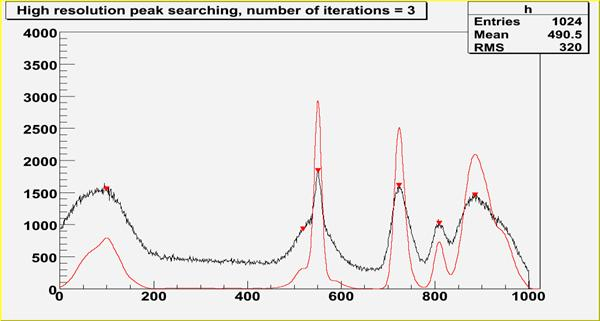Fig. 27 An example of one-dimensional synthetic spectrum with found peaks denoted by markers.

#### References:

1. M.A. Mariscotti: A method for identification of peaks in the presence of background and its application to spectrum analysis. NIM 50 (1967), 309-320.
2. M. Morhac;, J. Kliman, V. Matouoek, M. Veselsky, I. Turzo.:Identification of peaks in multidimensional coincidence gamma-ray spectra. NIM, A443 (2000) 108-125.
3. Z.K. Silagadze, A new algorithm for automatic photopeak searches. NIM A 376 (1996), 451.

Examples of peak searching method:

The SearchHighRes function provides users with the possibility to vary the input parameters and with the access to the output deconvolved data in the destination spectrum. Based on the output data one can tune the parameters.

### Example 15 - script SearchHR1.C:

One-dimensional spectrum with found peaks denoted by markers, 3 iterations steps in the deconvolution.

#### Script:

void SearchHR1() {
Int_t i,nfound,bin;
const Int_t nbins = 1024;
Double_t xmax = nbins;
Double_t source[nbins], dest[nbins];
gROOT->ForceStyle();
TString dir = gROOT->GetTutorialDir();
TString file = dir+"/spectrum/TSpectrum.root";
TFile *f = new TFile(file.Data());
TH1F *h = (TH1F*) f->Get("back2");
h->SetTitle("High resolution peak searching, number of iterations = 3");
h->GetXaxis()->SetRange(1,nbins);
TH1F *d = new TH1F("d","",nbins,xmin,xmax);
h->Draw("L");
for (i = 0; i < nbins; i++) source[i]=h->GetBinContent(i + 1);
h->Draw("L");
TSpectrum *s = new TSpectrum();
nfound = s->SearchHighRes(source, dest, nbins, 8, 2, kTRUE, 3, kTRUE, 3);
Double_t *xpeaks = s->GetPositionX();
for (i = 0; i < nfound; i++) {
a=xpeaks[i];
bin = 1 + Int_t(a + 0.5);
fPositionX[i] = h->GetBinCenter(bin);
fPositionY[i] = h->GetBinContent(bin);
}
TPolyMarker * pm = (TPolyMarker*)h->GetListOfFunctions()->FindObject("TPolyMarker");
if (pm) {
h->GetListOfFunctions()->Remove(pm);
delete pm;
}
pm = new TPolyMarker(nfound, fPositionX, fPositionY);
pm->SetMarkerStyle(23);
pm->SetMarkerSize(1.3);
for (i = 0; i < nbins; i++) d->SetBinContent(i + 1,dest[i]);
d->SetLineColor(kRed);
d->Draw("SAME");
printf("Found %d candidate peaks\n",nfound);
for( i=0;i<nfound;i++) printf("posx= %f, posy= %f\n",fPositionX[i], fPositionY[i]);
}
int Int_t
Definition: RtypesCore.h:45
Option_t Option_t TPoint TPoint const char GetTextMagnitude GetFillStyle GetLineColor GetLineWidth GetMarkerStyle GetTextAlign GetTextColor GetTextSize void char Point_t Rectangle_t dest
virtual void SetMarkerColor(Color_t mcolor=1)
Set the marker color.
Definition: TAttMarker.h:38
virtual void SetMarkerStyle(Style_t mstyle=1)
Set the marker style.
Definition: TAttMarker.h:40
virtual void SetMarkerSize(Size_t msize=1)
Set the marker size.
Definition: TAttMarker.h:41
Double_t * fPositionX
[fNPeaks] X position of peaks
Definition: TSpectrum.h:28
Int_t fNPeaks
number of peaks found
Definition: TSpectrum.h:26
Double_t * fPositionY
[fNPeaks] Y position of peaks
Definition: TSpectrum.h:29
TArc a
Definition: textangle.C:12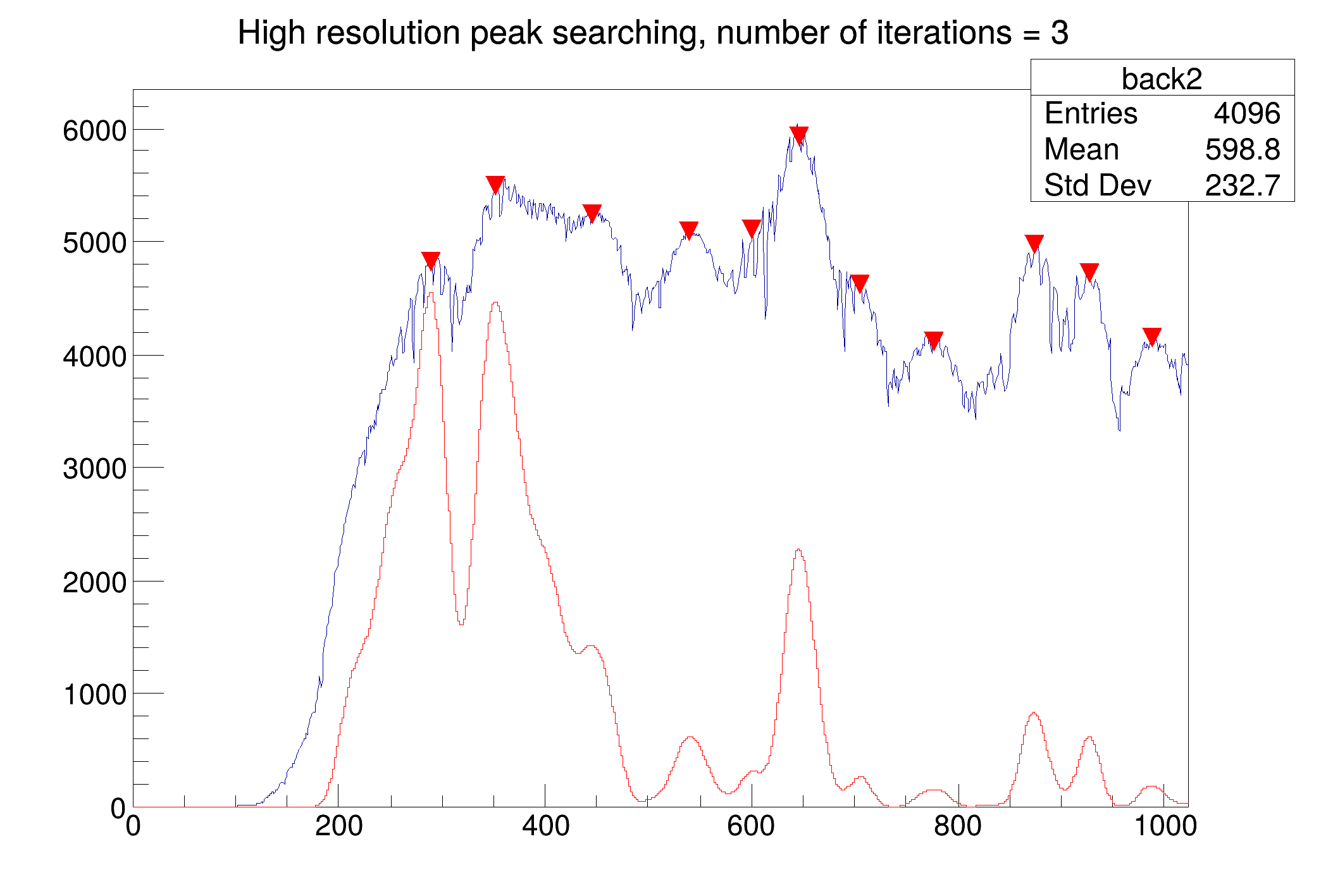### Example 16 - script SearchHR3.C:

Influence of number of iterations (3-red, 10-blue, 100- green, 1000-magenta), sigma=8, smoothing width=3.

void SearchHR3() {
Int_t i,nfound,bin;
const Int_t nbins = 1024;
Double_t xmax = nbins;
Double_t source[nbins], dest[nbins];
gROOT->ForceStyle();
TString dir = gROOT->GetTutorialDir();
TString file = dir+"/spectrum/TSpectrum.root";
TFile *f = new TFile(file.Data());
TH1F *h = (TH1F*) f->Get("back2");
h->SetTitle("Influence of # of iterations in deconvolution in peak searching");
h->GetXaxis()->SetRange(1,nbins);
TH1F *d1 = new TH1F("d1","",nbins,xmin,xmax);
TH1F *d2 = new TH1F("d2","",nbins,xmin,xmax);
TH1F *d3 = new TH1F("d3","",nbins,xmin,xmax);
TH1F *d4 = new TH1F("d4","",nbins,xmin,xmax);
TSpectrum *s = new TSpectrum();
for (i = 0; i < nbins; i++) source[i]=h->GetBinContent(i + 1);
nfound = s->SearchHighRes(source, dest, nbins, 8, 2, kTRUE, 3, kTRUE, 3);
Double_t *xpeaks = s->GetPositionX();
for (i = 0; i < nfound; i++) {
a=xpeaks[i];
bin = 1 + Int_t(a + 0.5);
fPositionX[i] = h->GetBinCenter(bin);
fPositionY[i] = h->GetBinContent(bin);
}
TPolyMarker * pm = (TPolyMarker*)h->GetListOfFunctions()->FindObject("TPolyMarker");
if (pm) {
h->GetListOfFunctions()->Remove(pm);
delete pm;
}
pm = new TPolyMarker(nfound, fPositionX, fPositionY);
pm->SetMarkerStyle(23);
pm->SetMarkerSize(1.3);
h->Draw("L");
for (i = 0; i < nbins; i++) d1->SetBinContent(i + 1,dest[i]);
d1->Draw("SAME");
for (i = 0; i < nbins; i++) source[i]=h->GetBinContent(i + 1);
s->SearchHighRes(source, dest, nbins, 8, 2, kTRUE, 10, kTRUE, 3);
for (i = 0; i < nbins; i++) d2->SetBinContent(i + 1,dest[i]);
d2->Draw("SAME");
for (i = 0; i < nbins; i++) source[i]=h->GetBinContent(i + 1);
s->SearchHighRes(source, dest, nbins, 8, 2, kTRUE, 100, kTRUE, 3);
for (i = 0; i < nbins; i++) d3->SetBinContent(i + 1,dest[i]);
d3->Draw("SAME");
for (i = 0; i < nbins; i++) source[i]=h->GetBinContent(i + 1);
s->SearchHighRes(source, dest, nbins, 8, 2, kTRUE, 1000, kTRUE, 3);
for (i = 0; i < nbins; i++) d4->SetBinContent(i + 1,dest[i]);
d4->Draw("SAME");
printf("Found %d candidate peaks\n",nfound);
}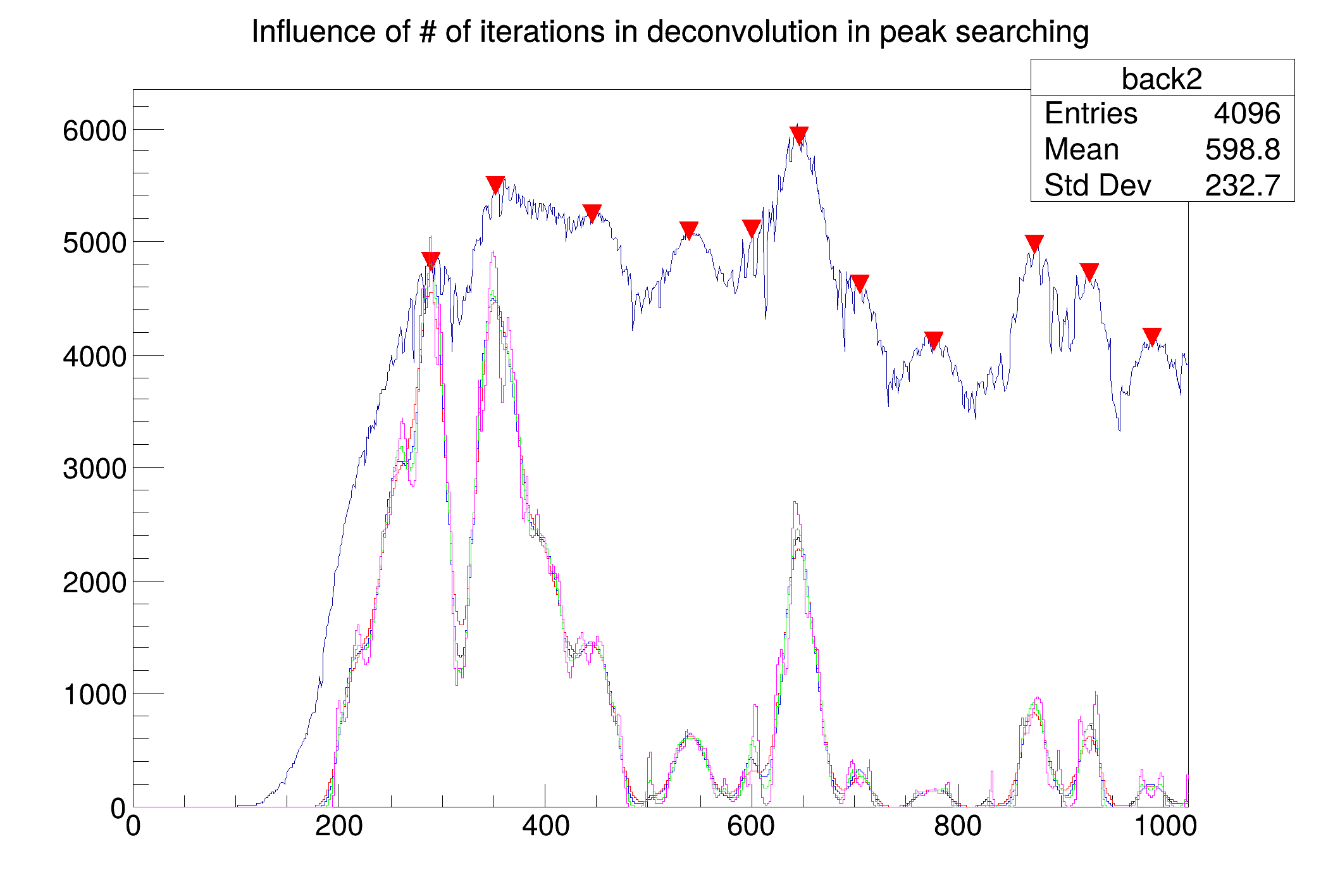Definition at line 2129 of file TSpectrum.cxx.

## ◆ SetAverageWindow()

 void TSpectrum::SetAverageWindow ( Int_t w = 3 )
static

Static function: Set average window of searched peaks (see TSpectrum::SearchHighRes).

Definition at line 102 of file TSpectrum.cxx.

## ◆ SetDeconIterations()

 void TSpectrum::SetDeconIterations ( Int_t n = 3 )
static

Static function: Set max number of decon iterations in deconvolution operation (see TSpectrum::SearchHighRes).

Definition at line 111 of file TSpectrum.cxx.

## ◆ SetResolution()

 void TSpectrum::SetResolution ( Double_t resolution = 1 )

NOT USED resolution: determines resolution of the neighbouring peaks default value is 1 correspond to 3 sigma distance between peaks.

Higher values allow higher resolution (smaller distance between peaks. May be set later through SetResolution.

Definition at line 354 of file TSpectrum.cxx.

## ◆ SmoothMarkov()

 const char * TSpectrum::SmoothMarkov ( Double_t * source, Int_t ssize, Int_t averWindow )

One-dimensional markov spectrum smoothing function.

This function calculates smoothed spectrum from source spectrum based on Markov chain method. The result is placed in the array pointed by source pointer. On successful completion it returns 0. On error it returns pointer to the string describing error.

#### Parameters:

• source: pointer to the array of source spectrum
• ssize: length of source array
• averWindow: width of averaging smoothing window

The goal of this function is the suppression of the statistical fluctuations. The algorithm is based on discrete Markov chain, which has very simple invariant distribution:

$U_2 = \frac{p_{1,2}}{p_{2,1}}U_1, U_3 = \frac{p_{2,3}}{p_{3,2}}U_2U_1, ... , U_n = \frac{p_{n-1,n}}{p_{n,n-1}}U_{n-1}...U_2U_1$

$$U_1$$ being defined from the normalization condition $$\sum_{i=1}^{n} U_i=1$$. $$n$$ is the length of the smoothed spectrum and

$p_{i,i\pm 1} = A_i\sum_{k=1}^{m} exp\left[ \frac{y(i\pm k)-y(i)}{y(i\pm k)+y(i)}\right]$

#### Reference:

1. Z.K. Silagadze, A new algorithm for automatic photopeak searches. NIM A 376 (1996), 451.

### Example 14 - script Smoothing.C

void Smoothing() {
Int_t i;
const Int_t nbins = 1024;
Double_t xmax = nbins;
Double_t source[nbins];
gROOT->ForceStyle();
TString dir = gROOT->GetTutorialDir();
TString file = dir+"/spectrum/TSpectrum.root";
TFile *f = new TFile(file.Data());
TH1F *h = (TH1F*) f->Get("back1");
h->SetTitle("Smoothed spectrum for m=3");
for (i = 0; i < nbins; i++) source[i]=h->GetBinContent(i + 1);
h->SetAxisRange(1,1024);
h->Draw("L");
TSpectrum *s = new TSpectrum();
TH1F *smooth = new TH1F("smooth","smooth",nbins,0.,nbins);
smooth->SetLineColor(kRed);
s->SmoothMarkov(source,1024,3); //3, 7, 10
for (i = 0; i < nbins; i++) smooth->SetBinContent(i + 1,source[i]);
smooth->Draw("L SAME");
}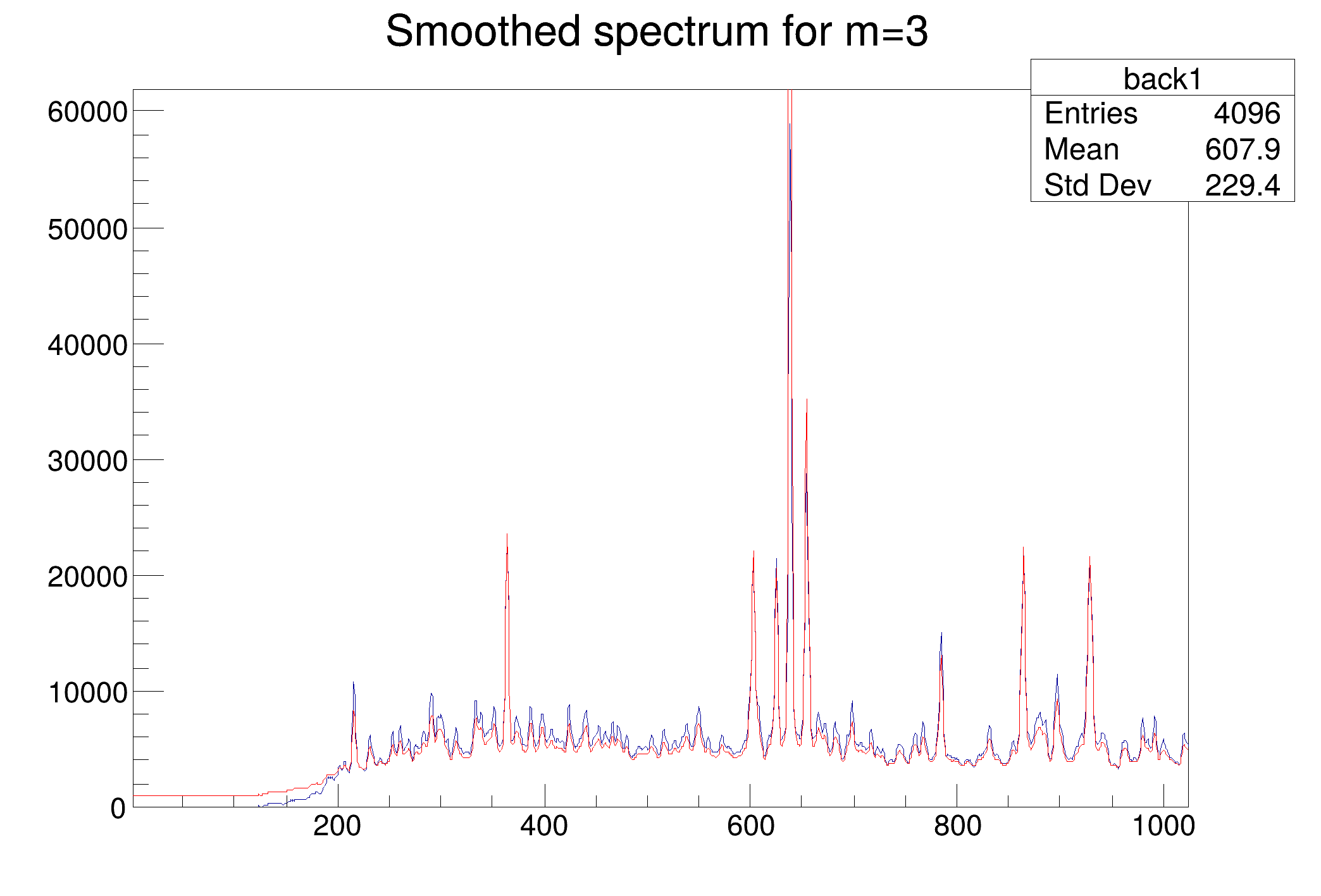Definition at line 1198 of file TSpectrum.cxx.

## ◆ StaticBackground()

 TH1 * TSpectrum::StaticBackground ( const TH1 * hist, Int_t niter = 20, Option_t * option = "" )
static

Static function, interface to TSpectrum::Background.

Definition at line 2590 of file TSpectrum.cxx.

## ◆ StaticSearch()

 Int_t TSpectrum::StaticSearch ( const TH1 * hist, Double_t sigma = 2, Option_t * option = "goff", Double_t threshold = 0.05 )
static

Static function, interface to TSpectrum::Search.

Definition at line 2580 of file TSpectrum.cxx.

## ◆ Streamer()

 void TSpectrum::Streamer ( TBuffer & R__b )
overridevirtual

Stream an object of class TObject.

Reimplemented from TNamed.

## ◆ StreamerNVirtual()

 void TSpectrum::StreamerNVirtual ( TBuffer & ClassDef_StreamerNVirtual_b )
inline

Definition at line 78 of file TSpectrum.h.

## ◆ Unfolding()

 const char * TSpectrum::Unfolding ( Double_t * source, const Double_t ** respMatrix, Int_t ssizex, Int_t ssizey, Int_t numberIterations, Int_t numberRepetitions, Double_t boost )

One-dimensional unfolding function.

This function unfolds source spectrum according to response matrix columns. The result is placed in the vector pointed by source pointer. The coefficients of the resulting vector represent contents of the columns (weights) in the input vector. On successful completion it returns 0. On error it returns pointer to the string describing error. If desired after every numberIterations one can apply boosting operation (exponential function with exponent given by boost coefficient) and repeat it numberRepetitions times. For details we refer to .

#### Parameters:

• source: pointer to the vector of source spectrum
• respMatrix: pointer to the matrix of response spectra
• ssizex: length of source spectrum and # of rows of the response matrix. ssizex must be >= ssizey.
• ssizey: length of destination coefficients and # of columns of the response matrix.
• numberIterations: number of iterations
• numberRepetitions: number of repetitions for boosted deconvolution. It must be greater or equal to one.
• boost: boosting coefficient, applies only if numberRepetitions is greater than one.

### Unfolding:

The goal is the decomposition of spectrum to a given set of component spectra.

The mathematical formulation of the discrete linear system is:

$y(i) = \sum_{k=0}^{N_y-1} h(i,k)x(k), i = 0,1,2,...,N_x-1$

$\begin{bmatrix} y(0) \\ y(1) \\ \dots \\ y(N_x-1) \end{bmatrix} = \begin{bmatrix} h(0,0) & h(0,1) & \dots & h(0,N_y-1) \\ h(1,0) & h(1,1) & \dots & h(1,N_y-1) \\ \dots \\ h(N_x-1,0) & h(N_x-1,1) & \dots & h(N_x-1,N_y-1) \end{bmatrix} \begin{bmatrix} x(0) \\ x(1) \\ \dots \\ x(N_y-1) \end{bmatrix}$

#### References:

1. Jandel M., Morhac; M., Kliman J., Krupa L., Matouoek V., Hamilton J. H., Ramaya A. V.: Decomposition of continuum gamma-ray spectra using synthesised response matrix. NIM A 516 (2004), 172-183.

### Example 13 - script Unfolding.C: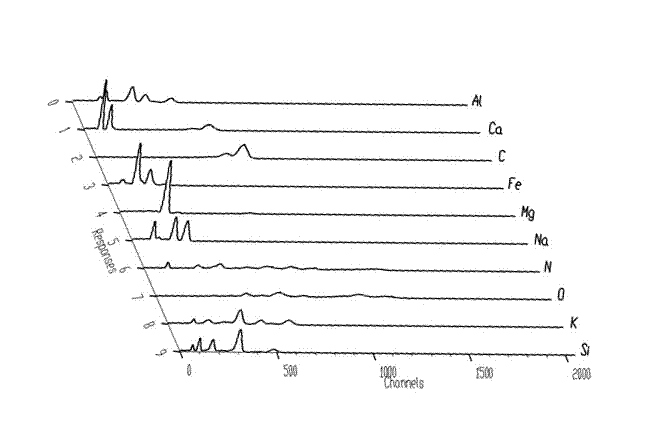Fig. 20 Response matrix composed of neutron spectra of pure chemical elements.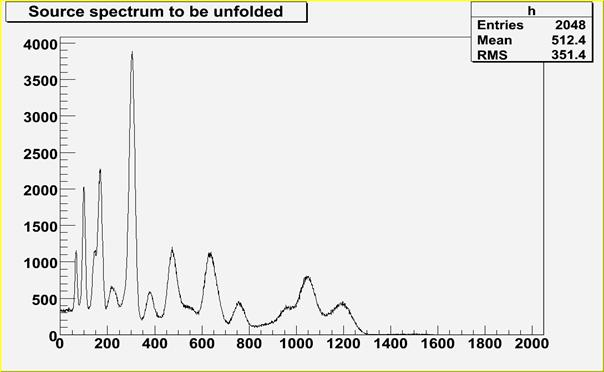Fig. 21 Source neutron spectrum to be decomposed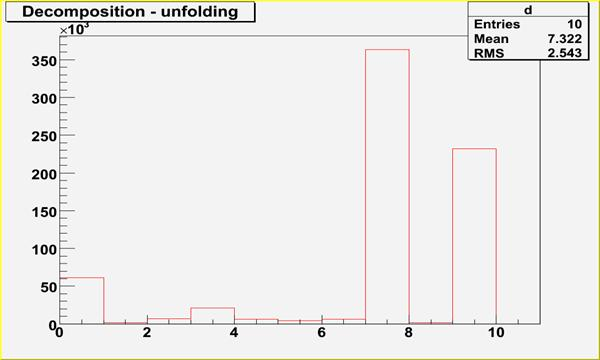Fig. 22 Spectrum after decomposition, contains 10 coefficients, which correspond to contents of chemical components (dominant 8-th and 10-th components, i.e. O, Si)

#### Script:

// Example to illustrate unfolding function (class TSpectrum).
// To execute this example, do
// root > .x Unfolding.C
void Unfolding() {
Int_t i, j;
Int_t nbinsx = 2048;
Int_t nbinsy = 10;
double xmin = 0;
double xmax = nbinsx;
double ymin = 0;
double ymax = nbinsy;
double *source = new double[nbinsx];
double **response = new double *[nbinsy];
for (i=0;i<nbinsy;i++) response[i] = new double[nbinsx];
TH1F *h = new TH1F("h","",nbinsx,xmin,xmax);
TH1F *d = new TH1F("d","Decomposition - unfolding",nbinsx,xmin,xmax);
TH2F *decon_unf_resp = new TH2F("decon_unf_resp","Root File",nbinsy,ymin,ymax,nbinsx,xmin,xmax);
TFile *f = new TFile("TSpectrum.root");
h = (TH1F*) f->Get("decon_unf_in;1");
TFile *fr = new TFile("TSpectrum.root");
decon_unf_resp = (TH2F*) fr->Get("decon_unf_resp;1");
for (i = 0; i < nbinsx; i++) source[i] = h->GetBinContent(i + 1);
for (i = 0; i < nbinsy; i++){
for (j = 0; j< nbinsx; j++){
response[i][j] = decon_unf_resp->GetBinContent(i + 1, j + 1);
}
}
TCanvas *Decon1 = (TCanvas *)gROOT->GetListOfCanvases()->FindObject("Decon1");
if (!Decon1) Decon1 = new TCanvas("Decon1","Decon1",10,10,1000,700);
h->Draw("L");
TSpectrum *s = new TSpectrum();
s->Unfolding(source,(const double**)response,nbinsx,nbinsy,1000,1,1);
for (i = 0; i < nbinsy; i++) d->SetBinContent(i + 1,source[i]);
d->SetLineColor(kRed);
d->SetAxisRange(0,nbinsy);
d->Draw("");
}
float ymin
Definition: THbookFile.cxx:95
float ymax
Definition: THbookFile.cxx:95
The Canvas class.
Definition: TCanvas.h:23
TObject * Get(const char *namecycle) override
Return pointer to object identified by namecycle.
2-D histogram with a float per channel (see TH1 documentation)}
Definition: TH2.h:257
Double_t GetBinContent(Int_t binx, Int_t biny) const override
Definition: TH2.h:89
const char * Unfolding(Double_t *source, const Double_t **respMatrix, Int_t ssizex, Int_t ssizey, Int_t numberIterations, Int_t numberRepetitions, Double_t boost)
One-dimensional unfolding function.
Definition: TSpectrum.cxx:1892

Definition at line 1892 of file TSpectrum.cxx.

## ◆ fgAverageWindow

 Int_t TSpectrum::fgAverageWindow = 3
staticprotected

Average window of searched peaks.

Definition at line 32 of file TSpectrum.h.

## ◆ fgIterations

 Int_t TSpectrum::fgIterations = 3
staticprotected

Maximum number of decon iterations (default=3)

Definition at line 33 of file TSpectrum.h.

## ◆ fHistogram

 TH1* TSpectrum::fHistogram
protected

resulting histogram

Definition at line 31 of file TSpectrum.h.

## ◆ fMaxPeaks

 Int_t TSpectrum::fMaxPeaks
protected

Maximum number of peaks to be found.

Definition at line 25 of file TSpectrum.h.

## ◆ fNPeaks

 Int_t TSpectrum::fNPeaks
protected

number of peaks found

Definition at line 26 of file TSpectrum.h.

## ◆ fPosition

 Double_t* TSpectrum::fPosition
protected

[fNPeaks] array of current peak positions

Definition at line 27 of file TSpectrum.h.

## ◆ fPositionX

 Double_t* TSpectrum::fPositionX
protected

[fNPeaks] X position of peaks

Definition at line 28 of file TSpectrum.h.

## ◆ fPositionY

 Double_t* TSpectrum::fPositionY
protected

[fNPeaks] Y position of peaks

Definition at line 29 of file TSpectrum.h.

## ◆ fResolution

 Double_t TSpectrum::fResolution
protected

NOT USED resolution of the neighboring peaks

Definition at line 30 of file TSpectrum.h.

Libraries for TSpectrum:[legend]

The documentation for this class was generated from the following files: